# KR100660958B1 - Successive approximation a/d converter - Google Patents

## Info

Publication number
KR100660958B1
KR100660958B1 KR1020050118484A KR20050118484A KR100660958B1 KR 100660958 B1 KR100660958 B1 KR 100660958B1 KR 1020050118484 A KR1020050118484 A KR 1020050118484A KR 20050118484 A KR20050118484 A KR 20050118484A KR 100660958 B1 KR100660958 B1 KR 100660958B1
Authority
KR
South Korea
Prior art keywords
circuit
potential
amplifier circuit
capacitance
output
Prior art date
Application number
KR1020050118484A
Other languages
Korean (ko)
Inventor
다츠오 가토
스구루 다치바나
가즈히로 미츠다
Original Assignee
후지쯔 가부시끼가이샤
Priority date (The priority date is an assumption and is not a legal conclusion. Google has not performed a legal analysis and makes no representation as to the accuracy of the date listed.)
Filing date
Publication date
Priority to JPJP-P-2005-00234724 priority Critical
Priority to JP2005234724A priority patent/JP4751667B2/en
Application filed by 후지쯔 가부시끼가이샤 filed Critical 후지쯔 가부시끼가이샤
Application granted granted Critical
Publication of KR100660958B1 publication Critical patent/KR100660958B1/en

• 239000003990 capacitor Substances 0.000 claims abstract description 144
• 230000000052 comparative effects Effects 0.000 claims abstract description 4
• 238000006243 chemical reaction Methods 0.000 description 62
• 230000001276 controlling effects Effects 0.000 description 4
• 230000000694 effects Effects 0.000 description 3
• 238000000034 methods Methods 0.000 description 2

## Images

•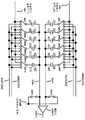•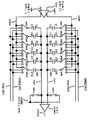•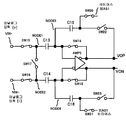••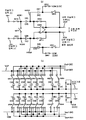•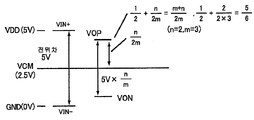••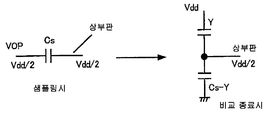•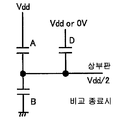•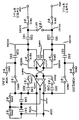•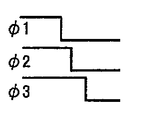•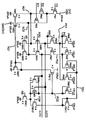•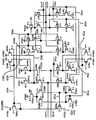•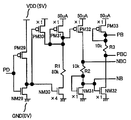••••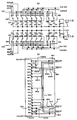•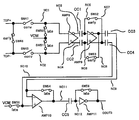•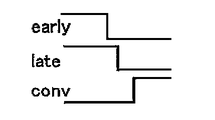••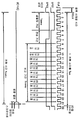•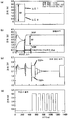•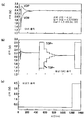•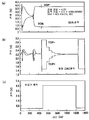•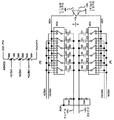•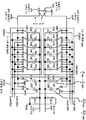## Classifications

• HELECTRICITY
• H03BASIC ELECTRONIC CIRCUITRY
• H03MCODING; DECODING; CODE CONVERSION IN GENERAL
• H03M1/00Analogue/digital conversion; Digital/analogue conversion
• H03M1/12Analogue/digital converters
• H03M1/34Analogue value compared with reference values
• H03M1/38Analogue value compared with reference values sequentially only, e.g. successive approximation type
• H03M1/46Analogue value compared with reference values sequentially only, e.g. successive approximation type with digital/analogue converter for supplying reference values to converter
• H03M1/466Analogue value compared with reference values sequentially only, e.g. successive approximation type with digital/analogue converter for supplying reference values to converter using switched capacitors
• H03M1/468Analogue value compared with reference values sequentially only, e.g. successive approximation type with digital/analogue converter for supplying reference values to converter using switched capacitors in which the input S/H circuit is merged with the feedback DAC array
• HELECTRICITY
• H03BASIC ELECTRONIC CIRCUITRY
• H03MCODING; DECODING; CODE CONVERSION IN GENERAL
• H03M1/00Analogue/digital conversion; Digital/analogue conversion
• H03M1/06Continuously compensating for, or preventing, undesired influence of physical parameters
• H03M1/0617Continuously compensating for, or preventing, undesired influence of physical parameters characterised by the use of methods or means not specific to a particular type of detrimental influence
• H03M1/0675Continuously compensating for, or preventing, undesired influence of physical parameters characterised by the use of methods or means not specific to a particular type of detrimental influence using redundancy
• H03M1/0678Continuously compensating for, or preventing, undesired influence of physical parameters characterised by the use of methods or means not specific to a particular type of detrimental influence using redundancy using additional components or elements, e.g. dummy components
• H03M1/068Continuously compensating for, or preventing, undesired influence of physical parameters characterised by the use of methods or means not specific to a particular type of detrimental influence using redundancy using additional components or elements, e.g. dummy components the original and additional components or elements being complementary to each other, e.g. CMOS
• H03M1/0682Continuously compensating for, or preventing, undesired influence of physical parameters characterised by the use of methods or means not specific to a particular type of detrimental influence using redundancy using additional components or elements, e.g. dummy components the original and additional components or elements being complementary to each other, e.g. CMOS using a differential network structure, i.e. symmetrical with respect to ground

## Abstract

SUMMARY OF THE INVENTION An object of the present invention is to provide a sequential comparison type AD converter having a sample hold amplifier circuit and capable of inputting and converting an AD signal having a signal amplitude equal to a power supply voltage.
The sequential comparison type AD converter is coupled to the output stage of the sample hold amplifier circuit and the sample hold amplifier circuit for sample-holding the input analog voltage and generating an internal analog voltage proportional to the input analog voltage with a voltage gain of less than one. A capacitor DA converter including a plurality of capacitors for accumulating charge according to the voltage, and switching the plurality of capacitive connections according to the control signal to generate an internal analog voltage and a comparative analog voltage according to the control signal at the output terminal; A comparator coupled to an output terminal of the comparator for generating a comparison result signal according to the comparison analog voltage at the output terminal, and a control circuit coupled to the output terminal of the comparator and supplying a control signal to the capacitive DA converter according to the comparison result signal.

## Description

SUCCESSIVE APPROXIMATION A / D CONVERTER}

1 is a diagram of a conventional charge redistribution differential capacitance DAC.

2 shows another circuit example of a conventional charge redistribution differential capacitance DAC.

3 is a diagram of a conventional sample hold amplifier circuit.

4 is a diagram showing a conventional sequential comparison type AD conversion circuit.

5 is a diagram showing an example of a sequential comparison type AD conversion circuit according to an embodiment of the present invention.

FIG. 6 shows the potential relationship of the circuit of FIG. 5; FIG.

7 is a diagram for explaining a capacitive DAC operation.

8 is a diagram for explaining a capacitive DAC operation.

9 is a diagram for explaining a capacitive DAC operation.

10 is a diagram of a charge transfer sample hold amplifier circuit suitable for the present invention.

FIG. 11 is a timing diagram showing the timing signal of FIG. 10; FIG.

12 is an illustration of an operational amplifier circuit (first stage) suitable for the circuit of FIG.

13 is an illustration of an operational amplifier circuit (second stage) suitable for the circuit of FIG.

14 is a circuit diagram showing an example of a bias circuit.

Fig. 15 is a diagram showing a circuit example for generating a voltage of about half of the power supply voltage.

16 is a diagram showing an example of a sequential comparison type AD conversion circuit according to an embodiment of the present invention.

17 shows an example of a sequential comparison type AD conversion circuit according to another embodiment of the present invention.

18 is a diagram showing an example of a sequential comparison type AD conversion circuit according to another embodiment of the present invention.

19 illustrates a comparator circuit suitable for the present invention.

20 is a timing diagram illustrating the timing signal of FIG. 19;

21 is a block diagram of a sequential comparison type AD conversion circuit of the present invention.

Fig. 22 is a diagram showing an example of the operation timing of the sequential comparison type AD conversion circuit of the present invention.

FIG. 23 is a diagram showing an example of operational waveforms of the circuit of FIG. 21; FIG.

FIG. 24 is a diagram showing an example of operation waveforms of the circuit of FIG. 21; FIG.

25 is a diagram showing an example of operation waveforms of the circuit of FIG. 21;

Fig. 26 is a diagram showing an example of a sequential comparison type AD conversion circuit according to another embodiment of the present invention.

27 shows an example of a sequential comparison type AD conversion circuit according to another embodiment of the present invention.

<Explanation of symbols for the main parts of the drawings>

SW13, SW14, SW15 to SW23, SW27 to SW42: switch

C25 to C28, C30 to C45: Capacity

AMP2: Amplifier Circuit

SHA1: sample hold amplifier circuit

CDAC1: Capacitive DA Converter

C0MP: Comparator

RDAC3: Resistor DA Converter

CNT: control circuit

The present invention relates to a sequential comparison type AD converter, and more particularly, to a sequential comparison type AD converter using a capacitive DA converter.

Sequentially comparable AD converters are realized with a relatively simple circuit configuration, are highly compatible with CMOS processes, can be manufactured relatively inexpensively, and can realize a medium conversion time and a medium conversion accuracy. It is used in. FIG. 1 shows a differential capacitance DAC of a conventional charge redistribution type AD converter as a representative configuration of a sequential comparison type AD converter (Patent Document 1 discloses the same circuit).

The configuration and operation of the circuit of FIG. 1 will be briefly described below. The circuit of FIG. 1 includes switches SW1 to SW14, capacitors C1 to C12, and comparator COMP1. VIN + represents the analog input of the positive side (+) of the differential input, and VIN- represents the analog input of the negative side (-) of the differential input. In addition, TOP + represents the positive side top plate of the capacitance array, and TOP − represents the negative side top plate of the capacitance array. Vref + is a reference potential (5V) on the positive side, and Vref- is a reference potential (0V) on the negative side. GND is the potential (2.5 V) of the signal ground of this circuit. In addition, VCM2 is the bias potential of the upper plate at the time of sampling, and COUT1 is the output of the comparator.

Doses C1 to C6 have doses of relative dose values of 1C, 1C, 2C, 4C, 8C and 16C, respectively. Similarly, the doses C7 to C12 have doses of relative dose values of 1C, 1C, 2C, 4C, 8C and 16C, respectively.

The state of the switch shown in FIG. 1 represents the state at the time of sampling. At the time of sampling, the potentials of VIN + are charged to the capacitors C1 to C6, and the potentials of VIN− are charged to the capacitors C7 to C12. After the end of sampling, the switches SW13 and SW14 are opened to switch the switches SW1 to SW12. By switching the switches SW1 to SW12, the potential of the lower plate (the electrode side connected to the switches SW1 to SW12) of the capacitors C1 to C12 is selectively set to one of Vref +, Vref-, and GND.

As a specific example, the plus side will be described. First, all of the capacitors C1 to C6 are charged to the analog input potential VIN + during sampling. After sampling, the switch SW13 is opened to make TOP + floating, for example, the capacitors C1 to C5 are connected to GND via the switches SW1 to SW5, and the capacitor C6 is connected via the switch SW6. It is connected to the quasi potential Vref +. By this connection, the electric charge accumulated by the input potential VIN + at the time of sampling is redistributed among the sampling capacitors C1 to C6, and the potential of TOP + becomes ((Vref +)-GND) / 2-VIN +. In this case, the potential is divided by half between Vref + and GND according to the total capacitance value of the capacitors C1 to C5 and the capacitance values of the capacitors C16 to 16C, and the potential obtained by subtracting the input potential VIN + from the divided voltage value. Becomes the potential of TOP +.

By controlling the connections of the switches SW1 to SW6, the potential of TOP + can be adjusted by changing the ratio of capacitive division between Vref + and GND. Similarly, by controlling the connections of the switches SW7 to SW12 on the negative side, the potential of the TOP − can be adjusted by changing the ratio of capacitive division between Vref − and GND. The comparator COMP1 inputs the potential of TOP + and the potential of TOP- as inputs, and produces the output COUT1 according to the difference of the positive potentials. The connection state of the switch is controlled so that a predetermined output is realized by sequentially changing the connection state of the switches SW1 to SW12 in accordance with the output COUT1. In this way, the digital code (state of the switch) corresponding to the difference between the analog input potentials VIN + and VIN- is searched by the control based on the output COUT1.

2 is a diagram illustrating another circuit example of the sequential comparison type AD converter. In FIG. 2, the same components as in FIG. 1 are referred to by the same numerals, and description thereof is omitted.

In the circuit configuration of Fig. 2, an amplifier AMP1 is provided on the input side. This amplifier AMP1 outputs amplification potentials IVINP and IVINN by amplifying analog input potentials VIN + and VIN-. By sampling these amplification potentials IVINP and IVINN in a capacitor array, the AD conversion operation as described above is executed. Using the circuit configuration shown in Fig. 2, the sampling operation can be made faster by charging the capacitors C1 to C12 through the amplifier AMP1 at the time of sampling.

Sequentially comparable AD converters are widely used for achieving medium conversion time and medium conversion accuracy, but pipelined AD converters are used for applications requiring higher speed (higher sampling rate) and low power. . In pipelined AD converters, a sample hold amplifier circuit is often used to sample the analog signal to achieve a high sampling rate.

3 is a diagram showing an example of the configuration of a conventional sample hold amplifier circuit. The same circuit is shown in the nonpatent literature 3.

The circuit of FIG. 3 includes switches SW15 to SW23, capacitors C13 to C16 and amplifier AMP2. VIN + represents the analog input potential on the positive side, and VIN− represents the analog input potential on the negative side. VOP is the positive output and VON is the negative output. NODE1 to NODE4 also represent internal nodes. BIAS1 is a bias potential of the bottom plate of the capacitors C15 and C16 at the time of sampling.

The state of the switch shown in FIG. 3 represents the state at the time of sampling. At the time of sampling, the potential of VIN + is charged to the capacitor C13, and the potential of VIN− is charged to the capacitor C14. In the hold state, the switches SW18, SW19, SW20, SW21 are opened, and the switches SW17, SW22, SW23 are closed. As a result, portions corresponding to the differential potentials of VIN + and VIN− of the charges accumulated in the capacitors C13 and C14 are transferred to the capacitors C15 and C16, and the differential potentials of the VIN + and VIN−, the positive side output VOP, and It is output as a negative side output VON.

The sample hold amplifier circuit as shown in FIG. 3 has achieved a high speed sampling operation as a pipelined AD converter.

In addition, a circuit configuration in which a function of a sample hold amplifier is incorporated in a sequential comparison type AD converter is also attempted. A circuit example of a sequential comparison type AD converter incorporating the function of the sample hold amplifier is shown in FIG. 4 (Patent Document 2).

The circuit of FIG. 4 includes switches SW24 to SW26, capacitors C17 to C24, and amplifiers AMP3 and AMP4. VIN is the analog input and COUT2 is the output of the comparator. NODE5 to NODE7 represent internal nodes, and BOT1 to BOT5 are bottom plates of capacity. In addition, Vdd / 2 represents the electric potential of 1/2 of a power supply voltage. In addition, as in the case of FIGS. 1 and 2, the value of nC (n is an integer) in parallel with the doses C17 to C24 indicates a relative size of each dose.

In the circuit of Fig. 4, an inverted amplifier circuit composed of capacitors C17 and C18 and amplifier AMP3 operates as a sample hold amplifier circuit. By providing this portion, it is not necessary to directly charge the capacitance of the capacitance DAC constituted by the capacitors C19 to C24 with an analog input signal, and by reducing the capacitance C17, it is possible to design a small input capacitance at VIN. do. As a result, the effect of speeding up the sampling can be obtained.

As circuit miniaturization proceeds, the demand for mounting the CM0S digital circuit and the CM0S analog circuit on the same chip is increasing. There is a demand for integrating CM0S analog circuits that have secured performance suitable for the high speed of fine CM0S digital circuits at the lowest possible cost.

For this reason, the demand for speed-up is increasing also for the sequential comparison type AD converter which realizes medium conversion time and medium conversion conversion accuracy with small area and low price. In order to speed up the sequential comparison type AD converter, it is important to speed up the judgment in the comparator and to shorten the sampling time.

However, for example, in the configuration as in the prior art shown in Fig. 1, since the sampling capacity is charged with an external analog input signal, the sampling time is limited by the capacity value of the sampling capacity, and it is difficult to shorten the sampling time sufficiently. In addition, in the configuration as in the conventional circuit of FIG. 2, charging is possible through the buffer amplifier at the time of sampling, thereby speeding up sampling but having a problem that an input signal exceeding the output signal range of the buffer amplifier cannot be sampled. In the most common circuit configuration, the voltage gain of the buffer amplifier is one. In this case, even if an input signal of the same voltage as the power supply voltage is input, the output signal amplitude of the buffer amplifier is not the same as the power supply voltage, resulting in a signal with a slight reduced voltage. For this reason, it was impossible to perform AD conversion by inputting the same signal amplitude as the power supply voltage.

In addition, the conventional sample hold amplifier circuit shown in FIG. 3 functions as a buffer amplifier of an analog input signal similarly to the conventional circuit of FIG. 2, and can speed up sampling. However, Non-Patent Document 3 merely shows an example of application to a pipelined AD converter, and does not mention the configuration, problems, and solutions in the case of application to a sequential AD converter.

In addition, in Patent Document 2, which discloses the conventional circuit of FIG. 4, the voltage gain of the amplifier is displayed only in the case of 1, and when AD conversion is required by inputting the same signal amplitude as the power supply voltage as in the conventional circuit of FIG. 2. Problem, the solution is not disclosed. In addition, the circuit configuration is shown only in the case of a single-ended switched capacitor type buffer amplifier, and there is no example of application to a differential circuit which is advantageous for noise generated from the digital circuit in the system LSI.

[Patent Document 1] US Patent No. 4,803,462

[Patent Document 2] Japanese Unexamined Patent Application Publication No. 10-336033

[Non-Patent Document 1] R. K. Hester et al., "Fully Differential ADC with Rail-to-Rail Common-Mode Range and Nonlinear Capacitor Compensation," IEEE Journal of Solid-State Circuits, Vol. 25, No. 1, pp. 173-183, Feb. 1990.

[Non-Patent Document 2] G. Promitzer, "12-bit Low-Power Fully Differential Switched Capacitor Noncalibrating Successive Approximation ADC with 1 MS / s," IEEE Journal of Solid-State Circuits, Vol. 36, No. 7, pp. 1138-1143, July 2001.

[Non-Patent Document 3] L. A. Singer et al., "A 14-Bit 10-NHz Calibration-Free CMOS Pipelined A / D Converter," Symposium on VLSI Circuits, pp. 94-95, 1996.

A first object of the present invention is to provide a sequential comparison type AD converter having a sample hold amplifier circuit for shortening a sampling time and capable of inputting and converting an AD signal having a signal amplitude equal to the power supply voltage.

It is also a second object of the present invention to provide a specific circuit configuration of the sample hold amplifier circuit described above.

Further, a third object of the present invention is to provide a double stage type DA converter suitable for a sequential comparison type AD converter corresponding to the first object.

A sequential comparison type AD converter according to the present invention comprises a sample hold amplifier circuit for sample-holding an input analog voltage and generating an internal analog voltage at an output terminal in proportion to the input analog voltage with a voltage gain of less than one; And a plurality of capacitors coupled to the output terminal for accumulating charges according to the internal analog voltage, and switching the connection of the plurality of capacitors according to a control signal to output the internal analog voltage and the comparative analog voltage according to the control signal. A comparator DA coupled to the output terminal of the capacitive DA converter, the comparator coupled to the output terminal of the capacitive DA converter, to generate a comparison result signal according to the comparison analog voltage, and coupled to the output terminal of the comparator. To supply a control signal to the capacitive DA converter Air is characterized in that it comprises a circuit.

Further, according to at least one embodiment of the present invention, a sample hold amplifier circuit having a voltage gain of less than 1 by making the value of the capacitance coupled to the output terminal larger than the capacitance coupled to the input terminal of the switched capacitor type sample hold amplifier circuit. To realize.

Further, according to at least one embodiment of the present invention, the double stage type DA converter is realized by combining the output of the resistor DA converter with the capacity of the minimum capacitance value among the plurality of capacitances of the capacitor DA converter.

(Example)

EMBODIMENT OF THE INVENTION Below, the Example of this invention is described in detail using attached drawing.

Fig. 5 is a circuit diagram showing a first embodiment of the sequential comparison type AD conversion circuit according to the present invention. FIG. 5A shows a sample hold amplifier circuit portion, and FIG. 5B shows a capacitor array portion connected to the output of the sample hold amplifier circuit portion. The output of this capacitive array portion is coupled to the comparator as in FIG.

The circuits shown in FIGS. 5A and 5B include switches SW13, SW14, SW15 to SW23, SW27 to SW42, capacitors C25 to C28, capacitors C30 to C45, and amplifier circuits. AMP2). VIN + is the analog input potential on the positive side, and VIN- is the analog input potential on the negative side. In addition, VOP and VON are internal analog signals on the plus and minus sides of the sample hold amplifier circuit output, respectively. TOP + represents the positive side top plate of the capacitor array, and TOP − represents the negative side top plate of the capacitor array. Vref + is the reference potential (5V) on the positive side, and Vref- is the reference potential (0V) on the negative side. In addition, VCM is a bias potential (for example, 2.5V) of the top plate at the time of sampling, and NODE1 to NODE4 are internal nodes. The value of kC (k is an integer) added to each dose Cn (n is an integer) indicates the relative magnitude of each dose value.

The state of the switch shown in Fig. 5A shows the sampling state of the sample hold amplifier circuit. The sampling state of the sample hold amplifier circuit will be described below.

By making the switches SW18 and SW19 closed, for example, the potentials of NODE3 and NODE4 are set to about 1/2 of the power supply voltage Vdd. In addition, the switches SW20 and SW21 are closed, and the bias potential VCM (2.5 V) of 1/2 of the power supply voltage Vdd is supplied to the lower plates of the capacitors C27 and C28. If the amplifier AMP2 is perfectly ideal and the offset is 0, the potentials of NODE3 and NODE4 are assumed to be 1/2 of Vdd. At this time, no charge is accumulated in the capacitors C27 and C28 (the charge accumulated in the C27 and C28 becomes zero). When the amplifier AMP2 is not ideal, the potentials of NODE3 and NODE4 do not coincide, and the potential difference is about the input conversion offset voltage of the amplifier AMP2. The potential of VCM is supplied to the lower plates of the capacitors C27 and C28 in order to store the offset voltage in the capacitors C27 and C28 and later eliminate the influence of the offset voltage.

At the same time, the switches SW15 and SW16 are closed to supply the potentials of VIN + and VIN− to the lower plates of the capacitors C25 and C26. When the amplifier AMP2 is perfectly ideal, the potentials of NODE3 and NODE4 become half of Vdd, so that electric charges corresponding to the potential difference between the capacitors C25 and C26 and VIN + and VIN- are accumulated.

When the potential of 1/2 of Vdd is represented by VCM, the charge Q25 accumulated in the upper plate of the capacitor C25 becomes as follows.

Q25 = -16C ((VIN +)-VCM)

Similarly, the charge Q26 accumulated in the upper plate of the capacitor C26 becomes as shown in Equation 2 below.

Q26 = -16C ((VIN-)-VCM)

Next, a state in which a voltage is extracted to the outputs VOP and VON by charge transfer will be described.

By operating the switch in the sampling state described above, charge is transferred to extract the desired output potential at the outputs VOP and VON. After storing the potential difference between VIN + and VIN- in the capacitors C25 and C26, the switches SW18 and SW19 are opened to make the NODE3 and NODE4 (top plates of the capacitors C25 to C28) floating. In addition, the switches SW20 and SW21 are opened to prevent the supply of the VCM potential to the lower plates of the capacitors C27 and C28. In addition, the switches SW17, SW22, SW23 are closed.

As a result, the potential of NODE1 and the potential of NODE2 become equal. Thus, the potential of NODE1 and the potential of NODE2 are represented by VCMB. In addition, the voltage gain of AMP2 is sufficiently large, and the potential of NODE3 and the potential of NODE4 can be approximated unless they change in the potential VCM at the time of sampling of the sample hold amplifier circuit. The potentials of NODE3 and NODE4 do not change in VCM, and the potentials of NODE1 and NODE2 become VCMB. In addition, since the charges of NODE3 and NODE4 are preserve | saved, the following formula (3) is established about the capacity | capacitance C25.

-16C ((VIN +)-VCM)

= -16C (VCMB-VCM) -24C (VOP-VCM)

In addition, the following formula (4) holds for the capacitor C26.

-16C ((VIN-)-VCM)

= -16C (VCMB-VCM) -24C (VON-VCM)

Here, the potential of the terminal VOP is represented by VOP, and the potential of the terminal VON is represented by VON. Subtracting Equation 4 from Equation 3 provides Equations 5 and 6 below.

-16C ((VIN +)-(VIN-)) = -24C (VOP-VON)

16 ((VIN +)-(VIN-)) / 24 = (VOP-VON)

That is, by the charge transfer from the capacitors C25 and C26 to the capacitors C27 and C28, the potential difference proportional to the difference voltage ((VIN +)-(VIN-)) of the analog input signal can be extracted to VOP and VON. have. In addition, the voltage gain can be made less than 1 by appropriately designing the sizes of the capacitors C25, C26, C27, and C28. In this example, 16/24 = 2/3, but in general, it can be of course (n / m, n <m). In addition, it is assumed that the common potentials of VOP and VON coincide with VCM.

In this way, by designing the voltage gain of the sample hold amplifier circuit to less than 1, saturation of the output of the amplifier AMP2 can be avoided even when the difference voltage of the analog input signal is equal to the power supply voltage. Thus, it becomes possible to handle analog input potential differences such as power supply voltages, that is, analog input signals in a rail-to-rail range.

The case where the amplifier AMP2 is not ideal and there is an offset will be described. The charge Q25 accumulated in the upper plate of the capacitor C25 at the time of sampling becomes the following formula (7).

Q25 = -16C ((VIN +)-VCMNODE3)

Here, VCMNODE3 is the potential of NODE3 at the time of sampling. Similarly, the charge Q26 accumulated in the upper plate of the capacitor C26 is expressed by the following expression (8).

Q26 = -16C ((VIN-)-VCMNODE4)

Here, VCMNODE4 is the potential of NODE4 at the time of sampling.

In this non-ideal situation, charges also accumulate on the upper plates of the capacitors C27 and C28. These charges Q27 and Q28 become the following expressions (9) and (10).

Q27 = 24C (VCMNODE3-VCM)

Q28 = 24C (VCMNODE4-VCM)

By operating the switch in this sampling state, charge is transferred to extract the desired output potential at the outputs VOP and VON.

From this, the potential of NODE1 and the potential of NODE2 become equal. Thus, the potential of NODE1 and the potential of NODE2 are represented by VCMB. In addition, the voltage gain of the amplifier AMP2 is sufficiently large, and the potential of NODE3 and the potential of NODE4 can be approximated if they do not change from the potentials VCMNODE3 and VCMNODE4 at the time of sampling of the sample hold amplifier circuit.

The potentials of NODE3 and NODE4 do not change from VCMNODE3 and VCMNODE4, and the potentials of NODE1 and NODE2 are VCMB. In addition, since the charges of NODE3 and NODE4 are preserve | saved, following formula (11) is established about NODE3.

-16C ((VIN +)-VCMNODE3) + 24C (VCMNODE3-VCM)

= -16C (VCMB-VCMNODE3) -24C (VOP-VCMNODE3)

In addition, the following expression (12) is established for NODE4.

-16C ((VIN-)-VCMNODE4) + 24C (VCMNODE4-VCM)

= -16C (VCMB-VCMNODE4) -24C (VON-VCMNODE4)

In addition, the potential of the terminal VOP is represented by VOP, and the potential of the terminal VON is represented by VON.

By subtracting equation (12) from equation (11), the following equations (13) and (14) can be obtained.

-16C ((VIN +)-(VIN-)) + 16C × VCMNODE3 + 24C × VCMNODE3

-16C X VCMNODE4 -24C X VCMNODE4

= 16C × VCMNODE3 + 24C × VCMNODE3-16C × VCMNODE4-24C × VCMNODE4-24C (VOP-VON)

16 ((VIN +)-(VIN-)) / 24 = (VOP-VON)

That is, even in the case of non-ideal offset, if the voltage gain of the amplifier AMP2 is large enough, the difference voltage ((VIN +) − The potential difference proportional to (VIN-)) can be extracted with VOP and VON.

As described above, the sample hold amplifier circuit transfers a potential difference proportional to the difference voltage ((VIN +) − (VIN−)) of the analog input signal to the outputs VOP and VON of the sample hold amplifier circuit. The potential difference between the outputs VOP and VON is further sampled with the capacitance DAC shown in Fig. 5B.

The state of the switch shown in Fig. 5B shows a state in which the sample hold amplifier circuit outputs VOP and VON are sampled by the capacitive DAC. The operation of sampling the sample hold amplifier circuit outputs VOP and VON with the capacitive DAC will be described below.

The switches SW27 to SW34 are all controlled such that the lower plate of the capacitors C30 to C37 leads to the VOP. In addition, the switch SW13 is closed, and VCM is supplied to the upper plates TOP + of the capacitors C30 to C37. That is, charges corresponding to the difference voltage between VCM and VOP are stored in the capacitors C30 to C37.

In addition, the switches SW35 to SW42 are controlled such that the lower plate of the capacitors C38 to C45 is connected to VON. The switch SW14 is closed and VCM is supplied to the top plate TOP- of the capacitors C38 to C45. In other words, charges corresponding to the difference voltage between VCM and VON are stored in the capacitors C38 to C45.

After the end of the sampling operation described above, the switches SW13 and SW14 are opened to switch the switches SW27 to SW32 and the switches SW35 to SW40 so that the lower plates of the capacitors C30 to C35 and the capacitors C38 and C43 [ The potential on the electrode side leading to SW27 to SW32 and SW35 to SW40 is selectively coupled to either Vref + or Vref-. Thereby, the digital code corresponding to the sampled analog potential difference ((VIN +)-(VIN-)) is searched.

As described above, since the voltage gain is less than 1 in the sample hold amplifier circuit shown in Fig. 5A, the decrease in potential difference due to this must be corrected. This correction will be described below.

In addition, in order to simplify description, the case where the power supply voltage Vdd is 5V, the reference voltage Vref + is 5V, Vref- is 0V, VCM is 2.5V, VIN + = 5V, VIN- = 0V is demonstrated. Even when the potential difference between the reference voltages Vref + (5 V) and Vref-(0 V), that is, the same potential difference as the power supply voltage (5 V) is input to VIN + and VIN-, the output of the amplifier AMP2 in FIG. In the example of FIG. 5, the voltage gain was 2/3 (= n / m). For this reason, as shown in FIG. 6, the sample hold amplifier circuit outputs VOP and VON ideally become the signal amplitude of 2/3 of a power supply voltage centering on bias potential VCM.

In the case of VIN + = 5V and VIN = 0V, as shown in FIG. 6, the potential of VOP is (1/2 + n / 2 m) x Vdd = 5 Vdd / 6 (n = 2, m = 3). do. Similarly, the potential of VON is Vdd / 6, and the potential difference is 2 Vdd / 3. When sampling the capacitive DAC, the potentials of the VOP and VON are sampled into the capacitive DAC. In the case of Vref + (5V) = Vdd = VIN +, FIG. 7 shows an outline of the operation of the positive side capacitance DAC for sampling the potential of the VOP.

7 shows the operation of the positive side capacitance DAC in the case of VIN + = 5V. At the time of sampling, the top plate (TOP +) of the capacitor DAC has VCM = 2.5V. In addition, the lower plate is 5 Vdd / 6. Cs of FIG. 7 shows the sum of the sampling capacities (C30 to C37 in FIG. 5) of the plus-side capacitance DAC.

After the end of the sampling, the potential of the lower plate is set to Vref + (5V) or Vref- (OV), so that the digital code whose potential of the lower plate becomes VOP is searched equivalently, and this is regarded as AD conversion result. In the right part of Fig. 7, the letter X denotes a capacitance portion of the total capacitance Cs to which the lower plate is connected to Vref + (5 V) = Vdd at the end of conversion. Therefore, the capacitance portion to which the lower plate is connected to Vref- (OV) is Cs-X. At the end of this conversion, the potential of the upper plate of the positive-side capacitance DAC is designed to match the potential VCM = 2.5 V of the upper plate at the time of sampling.

The negative side DAC is designed to replace Vref + of the positive side capacitance DAC with Vref − and Vref − with Vref +, and perform symmetrical operation about the VCM with respect to the potential. As a result, it is possible to design such that the potential TOP + of the upper plate of the positive-side capacitor DAC coincides with the potential TOP- of the upper plate of the negative-side capacitor DAC at the end of conversion.

In FIG. 7, when X is smaller than the final value, the potential of the positive side top plate is smaller than the final value, and the potential of the negative side top plate becomes larger than the final value. Therefore, the difference voltage can be detected by a comparator, and X can be made larger in the next comparison cycle to bring the upper plate potential difference closer to zero at the final value. This operation is executed by inputting TOP + and TOP-in FIG. 5 into the comparator to determine the magnitude relationship.

In FIG. 7, when X is larger than the final value, the potential of the positive side top plate is larger than the final value, and the potential of the negative side top plate is smaller than the final value. Therefore, by detecting the difference voltage with a comparator and making X smaller in the next comparison cycle, the upper plate potential difference can be made close to zero at the final value. In this way, the AD conversion value can be determined by searching for the final capacity distribution while detecting the top plate potential difference.

FIG. 8 is a diagram showing an outline of the operation of the positive-side capacitance DAC in the case of VIN + = VIN- = VCM. In the case of VIN + = VIN- = VCM, since VOP = VON = VCM = Vdd / 2 = 2.5V, the lower plate of the capacitance DAC at the time of sampling becomes Vdd / 2. The upper plate (TOP +) of the capacitance DAC at the time of sampling is VCM = 2.5V.

The right part of Fig. 8 shows the connection relationship of the capacitance at the end of conversion, and the symbol Y denotes the capacitance portion at which the lower plate is connected to Vref + (5 V) = Vdd at the end of conversion among the sum of the capacitances (Cs). . Therefore, the capacitance portion where the lower plate is connected to Vref- (OV) is Cs-Y. At the end of this conversion, the potential of the upper plate of the positive-side capacitance DAC is designed to match the upper plate potential VCM = 2.5 V at the time of sampling. The negative side DAC is designed to replace Vref + of the positive side DAC with Vref − and Vref − with Vref +, and to perform symmetrical operations about the VCM with respect to the potential as described above.

It can be seen from FIG. 8 that Y = Cs / 2 in the case of voltage gain n / m = 2/3 (n = 2, m = 3). 9 generally shows the capacity details of the plus-side capacity DAC.

9A does not depend on the comparison input code, and shows the capacitance portion where the lower plate is connected to Vref + (5V) = Vdd. FIG. 9B shows the capacitive portion in which the lower plate is connected to Vref-0V without depending on the comparison input code. The remaining D represents the capacitive portion where the bottom plate is connected back to Vref + (5 V) = Vdd or Vref-= OV, depending on the comparison input code.

By comparing FIG. 9, FIG. 8, and FIG. 7, A, B, and D of FIG. 9 can be determined. 8 shows the case of VIN + = VIN− = VCM, the potential difference between VIN + and VIN− is zero. Thus, the final input code of the plus side capacitive DAC is zero for all bits. That is, when the potential difference between VIN + and VIN- is 0, all of D in FIG. 9 are connected to Vref-0V. From Fig. 8, since Y = Cs / 2, A = Cs / 2.

On the other hand, as shown in FIG. 7, in the case of VOP = 5 Vdd / 6, X = 5 Cs / 6. For A = Cs / 2 and VOP = 5 Vdd / 6, we can determine B = Cs / 6 because the final input code of the plus-side capacitive DAC is designed to be full scale (all bits 1). Thus, in FIG. 9, depending on the capacitive DAC input code, the capacitive portion D whose bottom plate is connected back to Vref + (5V) = Vdd or Vref− = 0V is Cs-Cs / 2-Cs / 6 = Cs / 3.

Here, the flow returns to FIG. In FIG. 5, the case where the capacity DAC is 5 bits is shown as an example. When realizing a 5-bit capacitive DAC added to the most basic binary, as shown in Fig. 5, it is common to set C30 and C31 to 1C, C32 to 2C, C33 to 4C, C34 to 8C, and C35 to 16C. . Since this portion is a portion equivalent to D in FIG. 9, the total capacitance 32C of this portion is equal to Cs / 3 obtained as described above, that is, Cs / 3 = 32C, whereby Cs = 96C, A = The capacity of each part can be determined by Cs / 2 = 48C and B = Cs / 6 = 16C.

Capacitive portion A = 48C in which the lower plate is connected to Vref + (5V) = Vdd without depending on the comparison input code thus determined is indicated as the capacitor C36 in FIG. In addition, the capacitance portion B = 16C, to which the lower plate is connected with Vref-= O V, without being dependent on the comparison input code, is indicated by the capacitance C37.

After sampling the potentials of VOP and VON, the switches SW13 and SW14 are opened, the TOP + and the TOP- are suspended, and the comparison operation in the comparator is started. At this time, the switch SW33 is operated to couple the lower plate of the capacitor C36 to Vref + (5V), and the switch SW34 is operated to couple the lower plate of the capacitor C37 to Vref- = 0V. On the negative side, the lower plate of the capacitor C44 is coupled to Vref-0 V, and the lower plate of the capacitor C45 is coupled to Vref + (5 V).

Corresponding to the input code of the capacitor DAC, the lower plate of the capacitors C30 to C35 is selectively coupled to Vref + (5V) or Vref− (0V). For example, when the input code of the capacitor DAC is 01000, the lower plate of the capacitors C35, C33, C32, C31, and C30 is coupled to Vref − = 0 V, and the lower plate of the capacity C34 is coupled to Vref + (5 V). . In symmetry with this operation, in the negative side DAC, when the input code of the capacitor DAC is 01000, the lower plate of the capacitors C43, C41, C40, C39, and C38 is Vref + (5 V) and the lower plate of the capacitor C42 is Vref. To-(0 V).

While sequentially changing the input code to this DAC, the digital code whose minimum difference in potential between TOP + and TOP- is searched is searched. Let the value of the retrieved digital code be the AD conversion result.

As described in the process of finding A and B in Fig. 9, in the case of VIN + = 5 V and VIN-0 V, the potential of VOP is (1/2 + n / 2 m) x Vdd = 5 Vdd / 6 (n = 2 , m = 3). When the capacitor C36 is configured as 48 C, the capacitor C37 is configured as 16 C (and the capacitor C44 is 48 C, and the capacitor C45 is 16 C), the lower plates of the capacitors C31 to C35 are Vref + (5 V) and the capacitors C39 to C43 are Vref-. By coupling to (0 V), the potentials of TOP + and TOP- become almost the same. Therefore, even when the voltage gain of the sample hold amplifier circuit is n / m = 2/3, the digital conversion value 11111 can be obtained.

This is because the capacitors C36, C37, C44, and C45 operate as a scaling circuit for converting (maximum) values of VOP and VON to full scale (voltage) in the capacitor DAC. Similarly, in the case of VIN + = VIN-= VCM, it can be shown that the operation is performed exactly as described above.

As described above, in the circuit of FIG. 5, a sample hold amplifier circuit having a voltage gain of less than 1 and a capacitance DAC for sampling the potentials of the outputs VOP and VON are provided, and a reference voltage (Vref + and The capacitors C36, C37, C44, and C45 are provided for adjusting (scaling) the AD converter digital output so that the sample hold amplifier output at the time of inputting the Vref-potential difference) becomes the maximum value of the digital output of the AD converter. This makes it possible to supply a potential difference proportional to the analog input potential difference from the amplifier circuit to the capacitor DAC even when the reference voltage (potential difference between Vref + and Vref −) and the analog input potential difference are the same as the power supply voltage, and have a voltage gain of less than one. The correct digital output can be obtained by calibrating.

In addition, by configuring the sample signal by the sample hold amplifier circuit, the input capacitance of the sample hold amplifier circuit can be designed independently of the capacitor DAC. Therefore, by making this input capacitance small, it becomes possible to speed up a sequential comparison type AD conversion circuit.

In the description of Fig. 5, an example in which the voltage gain is n / m = 2/3 is shown, but it is clear that the circuit can be configured on the same principle in the case of other voltage gains.

FIG. 10 is a diagram showing an example of a specific circuit configuration from the sample hold amplifier circuit of FIG. 5A. In Fig. 10, the same components as in Fig. 5A are referred to by the same numerals and the description thereof is omitted.

The circuit of FIG. 10 includes switches SW15 to SW23, switches SW43 to SW46, capacitors C25 to C28, capacitors C46 to C49, and amplifier circuits AMP5 and AMP6. VIN + represents the analog input on the positive side, and VIN- represents the analog input on the negative side. VOP and VON are the positive side and the negative side (internal analog signal (+) and internal analog signal (-)) of the sample hold amplifier circuit output, respectively, and VCM is the bias potential of the lower plate of the capacitors C27 and C28 at the time of sampling. For example 2.5 V). NODE1 to NODE4, NODE8, and NODE9 are internal nodes, and vocm is a common potential of VOP and VON. In addition, phi 1, phi 2, and phi 3 are timing signals shown in FIG. 11, and control the opening and closing of the corresponding switch. The value of kC (k is an integer) added to each dose Cn (n is an integer) indicates the relative magnitude of each dose value.

In Fig. 5A, the amplifier circuit is shown as a single amplifier AMP2. However, as shown in Fig. 10, the amplifier circuit can be divided into AMP5 and AMP6. The state of the switch shown in FIG. 10 shows the state of the switch at the time of sampling the potential of VIN + and VIN-. The state at the time of this sampling is demonstrated first.

At the time of sampling, Φ1 is H, the switch with Φ1 and Φ2 is closed, and the switch with / Φ3 is open. As described in the description of Fig. 5A, at the time of sampling, SW15 and SW16 are closed and the potentials of VIN + and VIN− are supplied to the lower plates C25 and C26, NODE1 and NODE2. The difference between the circuits of FIG. 5 and FIG. 10 is that the potentials of NODE3 and NODE4 are the outputs of AMP5 and NODE8 and NODE9 by SW18 and SW19. The operation of storing the offset voltage by supplying the output of the amplifier to the input by SW18 and SW19 has been explained in FIG. When feeding the output of the amplifier to the input, a feedback loop is formed, so its gain and phase characteristics must be designed to be stable against oscillation. In the description of FIG. 5, the description has been made assuming that the voltage gain of AMP2 is sufficiently large. However, it may be difficult to achieve both a sufficiently large voltage gain and stability of the loop.

In this case, as shown in FIG. 10, AMP2 of FIG. 5 may be divided into AMP5 and AMP6 to be designed. When the output potential is transferred to VOP and VON, the voltage gain of the portion corresponding to AMP2 in Fig. 5 must be sufficiently large, so the circuit is designed so that the overall gain is determined by multiplying the voltage gain of AMP5 and AMP6. In the feedback operation of supplying potentials to NODE3 and NODE4, the output of AMP5 is supplied to NODE3 and NODE4 from SW18 and SW19. In this way, the gain and phase characteristics of AMP5 should be stable with respect to feedback during sampling, and the stability of the loop can be realized regardless of the gain and phase characteristics of AMP6.

In the circuit of Fig. 10, since the output of AMP5 having a relatively small gain (relative to AMP2 in Fig. 5) is supplied to NODE3 and NODE4 by SW18 and SW19, it is easy to ensure the stability of the loop. By performing feedback control so that the common potentials of NODE8 and NODE9 are close to VCM, the potentials of NODE8 and NODE9 at the time of sampling become VCM in an ideal state (this detail will be described later).

At the time of sampling of the sample hold amplifier circuit, since the potentials of NODE8 and NODE9 become almost VCM, the values of VOP and VON also become almost VCM (the values of VOP and VON are also feedback-controlled so that their common potential becomes VCM). box). Since SW45 and SW46 are closed, the center potential of the potential of VOP and VON is supplied to vocm.

Hereinafter, the potential output operation to VOP and VON after the end of sampling in the circuit of FIG. 10 will be described.

After supplying approximately VCM potentials to NODE3 and NODE4 to store charges in C25, C26, C27 and C28, first, SW18 and SW19 are opened. As a result, NODE3 and NODE4 become suspended, and the charges of NODE3 and NODE4 are preserved. Next, SW20 and SW21 are opened, and SW45 and SW46 are also opened. By opening SW45 and SW46, the potential of vocm is given a potential obtained by capacitively dividing the potentials of VOP and VON into C46 and C47, that is, the common potential of VOP and VON (capacities of C46 and C47 are equally equal to the upper plate at vocm, respectively). Connected).

SW17 is closed, and SW43, SW44, SW22, SW23 are closed. By closing SW43 and SW44, C48 and C49 are connected between the first stage outputs of the amplifier, NODE8 and NODE9, VOP and VON. These capacities work just like the mirror compensation capacities of a two-stage amplifier and act as mirror capacities to ensure loop stability (C48 and C49 are shown here as 32C, but load capacities are taken into account to maintain loop stability). Of course). By closing SW22 and SW23, the charges of NODE3 and NODE4 are preserved, and the potentials of VOP and VON are determined such that the potentials of NODE3 and NODE4 are virtual ground points, as in the circuit of FIG.

As in the circuit of FIG. 10, by dividing and implementing AMP2 in FIG. 5 into AMP5 and AMP6, it is possible to obtain the advantage that the stability of the loop can be easily designed.

FIG. 12 shows a circuit example of the transistor level of AMP5 in FIG. The circuit of FIG. 12 includes PMOS transistors PM1 to PM12 and NMOS transistors NM1 to NM11. VDD is the positive power supply (e.g. 5 V), GND is 0 V, inp, inn is the input of the amplifier, out1m, out1p is the output of the amplifier, NB, NBC is the bias potential of the NMOS, PB, PBC is PM0S The bias potential of VCM denotes a common potential input for common mode feedback, and ND1, ND2, PD1, PD2, PG1, PG2, and NG1 denote internal nodes of the amplifier. Xd (d is an integer) in parallel with the MOS transistors represents a design example of a relative value of each MOS transistor size, and a value of current written in a branch of a circuit represents an example of design of a current.

In FIG. 12, the same code | symbol is shown to the circuit terminal and circuit element corresponded to the circuit terminal and circuit element of FIG. The functions required for AMP5 in FIG. 10 are to amplify the potential difference between input inp and inn, output them to out1m and out1p, and control the common potential of the output voltage (for example) to VCM. This operation is mainly described below.

The circuit of FIG. 12 consists of a circuit which combined the common mode feedback circuit with the folding cascode circuit. PM1, PM2, PM3, PM4, PM5, PM6, PM7, NM3, PM4, PM5, PM6 form a folded cascode, and the remaining part constitutes a common mode feedback circuit. The folding cascode circuit acts as a circuit portion that amplifies the potential difference between the input inp and inn and outputs it to out1m and out1p.

Since the folded cascode circuit itself is a general circuit, a detailed description thereof will be omitted and the operation of the added common mode feedback circuit will be described. A differential circuit is formed with NM10 which inputs output potentials out1m and out1p to the gates of NM8 and NM9 and inputs VCM to the gate. Since the potentials of out1m and out1p become almost the same at the time of sampling of the sample hold amplifier circuit, the potentials of out1m and out1p are the same. If the potentials of out1m and out1p and the potential of VCM are the same, the same current flows through PM10 and PM11. In the example of FIG. 12, a current of 100 mA flows respectively. Since 100 mA of current flows through PM11, 200 mA of current flows through PM8 and PM9. Since 100 mA of current flows through PM10, 200 mA of current flows through NM1 and NM2.

A 200 mA current is supplied from PM8 and PM9, and a 200 mA current flows through NM1 and NM2. Therefore, in this case, PM8, PM9, NM1, NM2 do not affect the potential of out1m and out1p. Since the current flows from the normal folding cascode circuit, the sizes of PM6, PM7, NM3, and NM4 are changed to increase by an increase in current.

When the potential of out1m and out1p is lower than that of VCM, a large current flows through NM10. For this reason, the current of PM8 and PM9 becomes larger than the current flowing through NM1 and NM2. Therefore, the potentials of out1m and out1p increase. On the contrary, when the potential of out1m and out1p is higher than the potential of VCM, the current flowing in NM10 decreases. That is, the current flowing through NM1 and NM2 becomes larger than the current of PM8 and PM9.

In this way, by the feedback action of the common mode potential, the circuit operates so that the common mode potentials of the output potentials out1m and out1p of the amplifier coincide with VCM. As explained above as an example, the circuit of AMP5 of FIG. 10 can be embodied by the circuit structure as shown in FIG. FIG. 13 shows a circuit example in which the AMP6 of FIG. 10 is embodied in an almost identical thinking manner.

The circuit of FIG. 13 includes PMOS transistors PM24 to PM28 and NMOS transistors NM12 to NM28. VDD is the positive power supply (eg 5 V), GND is 0 V, out1m, out1p is the input of the amplifier, out2m, out2p is the output of the amplifier, NB, NBC is the bias potential of the NMOS, PB, PBC is The bias potential of the PMOS, the VCM, the common potential input for common mode feedback, and the ND3, ND4, PD3, PD4, PG3, PG4, PG5, NG2, NG3, NG4 represent nodes inside the amplifier. Note that xd (d is an integer) in parallel with the M0S transistor indicates a design example of the relative value of the size of each M0S transistor, and a value of the current written in the branch of the circuit indicates a design example of the current.

In Fig. 13, the same symbols are given to the circuit terminals and the circuit elements corresponding to the circuit terminals and the circuit elements in Fig. 10. Part composed of PM13, PM14, PM15, PM16, NM12, NM13, NM14, PM19, PM20, PM21, NM15, NM16, NM17, NM18, PM22, PM23, PM24, PM25, NM19, NM21, NM20, NM22 Is a differential amplifier circuit that amplifies the input signals out1m and out1p and outputs them to out2m and out2p. To increase the supply of load current to the output, note that the push-pull configuration and that the input differential pairs are provided for the NMOS and PMOS respectively in response to the push-pull configuration make the circuit operation relatively Since it will be easily understood, the detailed description of this partial operation is omitted. The operation of the remaining circuit portions that operate as the common mode feedback circuit will be described below.

A differential circuit is formed with NM24 which inputs the common potential vocm of the output potential to the gate of NM23, and inputs VCM to the gate. If the potential of vocm and the potential of VCM are the same, the same current flows through PM26 and PM27. In the example of FIG. 13, 100 mA of current flows, respectively. Since 100 mA of current flows through PM27, 200 mA of current flows through PM17 and PM18. Since 100 mA of current flows through PM26, 200 mA of current flows through NM26 and NM27.

A current of PM17, PM18 to 200 mA is supplied, and a current of 200 mA flows through the NM26 and NM27. Therefore, in this case, PM17, PM18, NM26, and NM27 do not affect the potentials of the outputs out2m and out2p. If the potential of vocm is lower than that of VCM, a large current flows through NM24. For this reason, the current of PM17 and PM18 becomes larger than the current flowing through NM26 and NM27. Therefore, the potentials of out2m and out2p rise. Conversely, when the potential of vocm is higher than that of VCM, the current flowing in NM24 decreases. That is, the current flowing through NM26 and NM27 becomes larger than the current of PM17 and PM18.

In this way, by the feedback action of the common mode potential, the circuit operates so that the common mode potentials of the output potentials out2m and out2p of the amplifier coincide with VCM. As explained above as an example, the circuit of AMP6 of FIG. 10 can be embodied by the circuit structure as shown in FIG.

FIG. 14 is a diagram illustrating an example of a circuit for supplying a bias potential to the circuits of FIGS. 12 and 13. The circuit of FIG. 14 includes PMOS transistors PM29 to PM33, NMOS transistors NM29 to NM32 and resistors R1 to R3. VDD represents a positive power supply, GND represents 0 V, NB and NBC represent the bias potential of the NMOS, PB and PBC represent the bias potential of the PMOS, and PD represents the control signal input for power down. Xd (d is an integer) written in the MOS transistor indicates a design example of the relative value of each M0S transistor size, a current value written in a branch of the circuit designates an example of the current, and a resistance value written in the resistor designates a resistance value. For example.

The circuit of FIG. 14 operates as a bias circuit for supplying a potential to PB, PBC, NB, and NBC of the circuits of FIGS. 12 and 13.

The current flowing through the resistor R1 flows into the PM32 in the current mirror circuit, thereby generating NB. In addition, NB is level shifted by the resistor R2 (10 k x 50 mA = 500 mV in the example of FIG. 14) to generate a cascode bias NBC. Similarly, bias is generated for PB and PBC. It is possible to supply a bias potential to the amplifier circuits of FIGS. 12 and 13 by a bias circuit as shown in FIG. 14.

FIG. 15 shows an example of a bias circuit for supplying a bias potential VCM to the circuits of FIGS. 5, 10, 12, and 13. As described above, VCM is a bias potential which is set at about half of the power supply voltage Vdd. In principle, by dividing the power supply voltage by a resistor, a desired electric potential can be easily generated. However, as can be seen from the circuit configuration of FIG. 5, all the top plates during sampling of the capacitive DAC, and during sampling of the sample hold amplifier circuit, are shown. The lower plates of C27, C28, etc. should be charged to the potential of VCM, and in order to design the time constants of these nodes sufficiently small, the resistance of the resistor that divides the power supply voltage should be designed small enough, and there is a problem of increased power consumption. . Therefore, it is preferable to use the bias circuit as shown in Fig. 15 in order to reduce the equivalent resistance of the VCM while keeping the power consumption small.

The circuit of FIG. 15 includes PMOS transistors PM34 to PM37, NMOS transistors NM33 and NM34 and resistors R4 to R7. VDD represents a positive power supply, GND represents 0 V, VCM represents a bias potential of about Vdd 1/2, PD represents a control signal input for power down, and NODE10 represents an internal node. Incidentally, xd (d is an integer) written in the MOS transistor indicates a design example of the relative value of each M0S transistor size, and the resistance value written in the resistor indicates an example of the design of the resistance value.

The potential of NODE10 is set to approximately 1/2 of the power supply voltage by the resistors R4 and R5. The effective resistance of PM34 and PM35 shall be small enough. Since the current flowing through R6, NM33, PM36, and R7 is smaller than the current flowing through R4 and R5, the effect of R6, NM33, PM36, and R7 on NODE10 is small, and the potential of NODE10 is determined to be approximately 1/2 of the supply voltage. I think. Since the VCM outputs the potential of NODE10 to the source follower of the PMOS and the NMOS, respectively, the potential of the VCM is also set to approximately 1/2 of the power supply voltage. By using the push-pull source follow output, the steady current is small and the time constant at the time of charging can be designed.

FIG. 16 is a diagram illustrating a configuration of a circuit in which the sequential comparison type AD conversion circuit of FIG. 5 is generalized. In Fig. 16, the same components as in Fig. 5 are referred to by the same reference numerals.

The circuit of FIG. 16 includes switches SW13, SW14, SW27 to SW42, capacitors C30 to C45, and sample hold amplifier circuit AMP7. VIN + represents the analog input on the positive side, and VIN- represents the analog input on the negative side. VOP and VON are the plus side and the minus side (internal analog signal (+) and internal analog signal (-)) of the sample hold amplifier circuit output, respectively. TOP + denotes the positive side top plate of the capacitor array, and TOP − denotes the negative side top plate of the capacitor array. Vref + is a reference potential (5V) on the positive side, and Vref- is a reference potential (0V) on the negative side. VCM represents the bias potential (2.5 V) of the top plate at the time of sampling, CDAC + represents the positive side capacitance DAC, and CDAC − represents the negative side capacitance DAC. SCL1 is a circuit for scaling the AD converter digital output so that the sample hold amplifier output when the reference voltage (potential difference between Vref + and Vref −) is input to the sample hold amplifier circuit becomes the maximum value of the digital output of the AD converter. The value of kC (k is an integer) added to each dose Cn (n is an integer) indicates the relative magnitude of each dose value.

The amplifier AMP7 of FIG. 16 generally shows the sample hold amplifier circuits (AMP2, C25 to C28 and switches, etc.) of FIG. A switched capacitor type sample hold amplifier circuit capable of determining the voltage gain with a relative ratio of capacitance is preferable from the viewpoint of precision. However, there is no problem in operation even if an amplifier circuit which directly determines the voltage amplification ratio by the resistance ratio is used as the AMP7. The important point here is that the voltage amplification factor is less than one. If the voltage amplification factor is n / m (n <m) and the common potential of VOP and VON is controlled to match VCM, AMP7 has the same effect as that of the circuit of FIG. 5 regardless of the specific circuit configuration. You can get it.

That is, by designing the voltage gain of the amplifier AMP7 to less than 1, the output saturation of the amplifier AMP7 can be avoided even when the voltage difference of the analog input signal is equal to the power supply voltage, so that the analog input potential difference equal to the power supply voltage, that is, It can handle analog input signals in the rail-to-rail range. In addition, the capacitance (C36) for adjusting (scaling) the AD converter digital output so that the sample hold amplifier output when the reference voltage (potential difference between Vref + and Vref −) is input to the sample hold amplifier circuit becomes the maximum value of the digital output of the AD converter. , C37, C44, C45) (SCL1) are installed. Thus, even when the amplifier AMP7 having a voltage gain of less than 1 (n / m, n <m) is used, an appropriate digital output value (the same digital output value as when sampling an analog signal directly from the capacitive DAC) can be obtained.

In addition, by transmitting the analog signal to the capacitor DAC through the amplifier AMP7, the input capacitance of the amplifier AMP7 can be designed independently of the capacitor DAC. Therefore, by making this input capacitance small, it becomes possible to speed up a sequential comparison type AD conversion circuit.

In Fig. 16, A = n / m written together in the amplifier AMP7 represents the voltage gain of AMP7, and A = 2/3 in the case of the same design as in Fig. 5. When the total capacity of the positive side (or negative side) capacitance DAC is 32C under the condition of A = 2/3, it is shown in FIGS. 5, 6, and 7 that the capacitances C36 and C44 are 48C, and the capacitances C37 and C45 are 16C. 8 and 9 have been described. In the following, a method of determining the capacity for scaling is generalized and explained.

Similarly to the circuit of FIG. 5, the circuit of FIG. 16 is controlled so that the common potential of VOP and VON coincides with VCM. In addition, the amplifier AMP7 transfers a potential difference proportional to the difference voltage ((VIN +)-(VIN−)) of the analog input signal to VOP and VON, and also samples the potential difference with the capacitance DAC. The state of the switch shown in FIG. 16 shows a state in which VOP and VON are sampled by the capacitance DAC (plus side capacitance DAC, negative side capacitance DAC) similarly to the case of FIG.

6, the relationship between the voltage gain and the potential between VOP and VON will be described. The voltage gain of the amplifier AMP7 is n / m. For the sake of simplicity, the case where the power supply voltage Vdd is 5 V, the reference voltage Vref + is 5 V, Vref − is 0 V, VCM is 2.5 V, VIN + = 5 V, and VIN − = 0 V will be described.

The potential of the VOP is 1/2 + n / 2m = (m + n) / 2m (× Vdd) when the voltage gain of the amplifier AMP7 is n / m. Considering that the potential of the VOP in FIG. 7 is replaced by (m + n) / 2m, the upper plate potential of the capacitance DAC at full scale (when the input potential difference is the same as the power supply voltage) is Vdd / 2, and the lower plate potential is Vdd (m + n). / 2m.

The charge accumulated on the top plate is as shown in Equation 15 below.

-CsVdd ((m + n) / 2m-1 / 2) = -CsVdd × n / 2m

Assuming that this charge is preserved, X in FIG. 7 is obtained, and the capacitance connection ratio at the end of conversion at the full scale input is obtained as shown in Equation 16 below.

(-X + Cs-X) Vdd / 2 = -CsVdd × n / 2 m

Solving Equation 16, Equation 17 below

X = Cs (m + n) / 2 m

Can be obtained.

In addition, when the difference voltage ((VIN +)-(VIN-)) of the analog input signal is 0, VOP is equal to VCM, and Y = 1/2 (Cs / 2) is apparent (see Fig. 8).

Since B of FIG. 9 is the same as Cs-X of FIG. 7 (the portions corresponding to D of FIG. 9 are all connected to Vdd when the digital codes are all bit 1), the following formula (18)

B = Cs-X = Cs-Cs (m + n) / 2m = Cs (m-n) / 2m

Can be obtained. 8 is equal to B + D. This is because the portion corresponding to D in FIG. 9 is all connected to OV when the digital codes are all bits O. FIG. Therefore, Equation 20 can be obtained from Equation 19 below.

Cs / 2 = Cs (m-n) / 2m + D

D = Cs × n / 2 m

Here, reference is again made to FIG. 16. Since the relationship between the capacity (D) of the part where the lower plate is reconnected and the total capacity (Cs) is generally obtained from Equation 20 based on the digital code of the capacity DAC, for example, when the part of D is represented by CB, The capacity of is represented by Equation 21 below.

Cs = CB × 2m / n

Substituting this equation (21) into equation (18), the capacitance values of B, i.e., the capacitances C37 and C45 in FIG.

B = Cs (m-n) / 2 m

= CB × 2m / n × (m-n) / 2m

= CB × (m-n) / n

The result of expression (22) is also shown in FIG. In the case of CB = 32C, m = 3, n = 2, B = 16C, which corresponds to the values of the capacities C37 and C45 described in FIG.

In addition, Y = 1/2 of FIG. 8 is the same as A of FIG. This is because portions corresponding to D in FIG. 9 are all connected to OV when the digital codes are all bits O. FIG. therefore,

A = Cs / 2 = CB × m / n

Can be obtained. This corresponds to the capacitance values of the capacitors C36 and C44 in FIG. This result is also shown in FIG. In the case of CB = 32C, m = 3, n = 2, A = 48C, which corresponds to the values of the capacities C36 and C44 described in FIG.

In this way, when the voltage gain of the amplifier AMP7 is generally n / m, the magnitudes of the capacitances C36, C37, C44, and C45 of the scaling circuit SCL1 are changed to the positive side capacitance DAC and the negative side capacitance DAC. Can be determined based on the total dose of. Since the circuit sampling operation and digital value search operation of FIG. 16 are the same as those of the circuit of FIG. 5, description thereof is omitted.

Fig. 17 is a circuit diagram showing the construction of another embodiment of the sequential comparison type AD conversion circuit according to the present invention. The circuit of FIG. 17 includes switches SW13, SW14, SG3, SG4, SG1 and SG2 shown in FIG. 17A, capacitances C30 to C45, and resistor DAC RDAC1 shown in FIG. 17B. do. VOP and VON are the plus side and the minus side (internal analog signal (+) and internal analog signal (-)) of the sample hold amplifier circuit output, respectively. TOP + denotes the positive side top plate of the capacitor array, and TOP − denotes the negative side top plate of the capacitor array. Vref + is a positive side reference potential (5 V), Vref − is a negative side reference potential (0 V), and VCM is a bias potential (2.5 V) of the upper plate during sampling. Resistor DAC RDAC1 includes resistors RD0 to RD7 and selectors SEL1 and SEL2 and generates RDACP and RDACN. NRD1 to NRD7 are internal nodes of the resistor DAC. The value of kC (k is an integer) added to each dose Cn (n is an integer) indicates the relative magnitude of each dose value.

The state of the switch shown in FIG. 17 has shown the state which samples VOP and VON. In Fig. 17, circuit parts having the same functions as the circuits of Figs. 5 and 16 are referred to by the same reference numerals. The circuit of FIG. 17 differs from the circuits of FIGS. 5 and 16 in that the AD conversion circuit is formed only by the capacitor DAC in the circuits of FIGS. 5 and 16, whereas the upper bit is determined as the capacitor DAC in the circuit of FIG. 17. The circuit is configured to determine the lower bit as a resistor DAC. As shown in FIG. 17, the inventive concept can also be applied to a complex DAC consisting of capacitance and resistance.

In the circuits of FIGS. 5 and 16, when searching for a digital code corresponding to an analog input, the capacitors C31 to C are fixed while the lower plate of the capacitor C30 is fixed to Vref − and the lower plate of the capacitor C38 is fixed to Vref +. The lower plate of C35) is connected to Vref + if the corresponding bit of the digital code is 1, and to Vref- if the corresponding bit of the digital code is O. In the negative-side capacitance DAC, it is symmetrically connected to the positive-side capacitance DAC, and is connected to Vref-if the corresponding bit of the digital code is 1, and to Vref + if the corresponding bit of the digital code is O.

Therefore, for example, when 10 bits of resolution are required, there is a problem that 1024 unit capacities are required on only one side, and the occupying area becomes large. The problem of the occupied area can be solved by making the DAC a double stage DAC.

As illustrated in FIG. 17, the CR double stage DAC can be configured by connecting the resistor DAC output RDACP to the lower plate of the capacitor C30. The CR double stage DAC can also be configured for the negative side capacitance DAC by connecting the resistor DAC output DRACN to the lower plate of the capacitor C38 of the negative side capacitance DAC.

RDAC1 shows an example of a resistor DAC for converting the lower 3 bits, and divides the reference voltage (potential difference between Vref + and Vref−) by 8 into unit resistors RD0 to RD7 (signs from NRD1 to NRD7 in order from low potential). ). The selector SEL1 selects the potential of any one of Vref − and the internal nodes NRD1 to NRD7 of the resistor DAC by the input digital value and outputs it to the RDACP. For example, when the input digital value is 000, Vref-is outputted, and the values from NRD1 to NRD7 are outputted as the digital values increase sequentially.

The selector SEL2 selects one of the potentials of Vref + and the potentials of NRD7 to NRD1 by the input digital value and outputs it to the RDACN. For example, Vref + is output when the input digital value is OOO in symmetry with RDACP, and outputs values from NRD7 to NRD1 as the digital values increase sequentially.

As in the circuit shown in Fig. 17, even when the DAC is configured as a double stage DAC composed of capacitance and resistance, the relationship between the maximum values of VOP and VON and the necessary conversion result is not different from those in the circuits of Figs. The construction principle of the scaling circuit SCL1 described with reference to FIGS. 5 and 16 can be applied as it is. For example, in the circuit of Fig. 17, when the voltage gain of the circuits outputting VOP and VON is 2/3, the values of the capacitors C36 and C44 are 48C, and the values of the capacitors C37 and C45 are 16C.

As described above, even when the CR double stage DAC is used, the principles of the voltage gain and scaling circuit of the present invention can be applied as it is. Therefore, the advantages of the double stage DAC in which the occupied area is suppressed small and the advantage of the sequential comparison ADC of the present invention that the input capacitance can be kept small can be compatible.

Fig. 18 is a circuit diagram showing the construction of another embodiment of the sequential comparison type AD conversion circuit according to the present invention. The circuit of FIG. 18 includes switches SW13, SW14, SG5, SG6, SG1, SG2, capacitors C31 to C37, C39 to C45, C50 to C53, and FIG. 18B shown in FIG. The resistor DAC (RDAC2) shown in FIG. VOP and VON are the plus side and the minus side (internal analog signal (+) and internal analog signal (-)) of the sample hold amplifier circuit output, respectively. TOP + is the positive side top plate of the capacitance array, TOP − is the negative side top plate of the capacitance array, Vref + is the positive side reference potential (5 V), Vref − is the negative side reference potential (0 V), and VCM is the top plate at sampling Is the bias potential (2.5 V). The resistor DAC RDAC2 includes resistors RU1 to RU14, RH1 to RH8 and selectors SEL3, SEL4, SEL5, and SEL6. RDACUP, RDACUN, RDACLP, and RDACLN are the outputs of the resistor DACs, and VOFFP and VOFFN are bias potentials for offsetting the conversion results. Also, NRU1 to NRU4, NRU8, NRU12 to NRU15 represent internal nodes of the resistive DAC. The value of kC (k is an integer) added to each dose Cn (n is an integer) indicates the relative magnitude of each dose value.

The state of the switch shown in FIG. 18 shows the state of sampling VOP and VON. In Fig. 18, circuit parts that have the same function as the circuit parts of Figs. 5, 16, and 17 are referred to by the same reference numerals. In the circuit of FIG. 17, a circuit example of supplying the resistance DAC output to the lower plates of the capacitors C30 and C38 is shown. In the circuit of FIG. 18, instead of the capacitors C30 and C38, the capacitors C50, C51, C52, and C53 are used. The resistor DAC output is supplied to the lower plates of the capacitors C50, C51, C52, and C53. It consists of a circuit structure which adds each resistance DAC output by a capacitance by the capacitance C50, C51 or C52, C53.

First, the configuration of the resistor DAC will be described. RDAC2 of Figure 18 acts as a resistor DAC for converting the lower four bits. RU1 to RU14 and RH1 to RH8 represent unit resistances having the same resistance value. Since RH1 to RH4 are connected in series by connecting two unit resistors in parallel, eventually four resistors are equivalent to one unit resistor. Similarly with respect to RH5-RH8, four resistances are equivalent to unit resistance. Therefore, the reference voltage (potential difference between Vref + and Vref−) is divided into 16 equal parts between RU1 to RU14 and RH1 to RH8. The internal nodes of the resistor DAC are given the symbols NRUe (e is an integer) in order from the lower potential side. Here, e of NRUe corresponds to the potential of the reference potential e / 16 divided by sixteen.

VOFFN obtained by further dividing the potential difference between NRU15 and Vref + by two resistors RH5 to RH8 becomes a potential as low as ((Vref +)-(Vref −)) / 32 from Vref +. Similarly, VOFFP obtained by further dividing the potential difference between NRU1 and Vref-by two resistors RH1 to RH4 becomes a potential as high as ((Vref +)-(Vref −)) / 32 from Vref-.

RDAC2 inputs a 4-bit digital signal to output potentials corresponding to the upper two bits to RDACUN and RDACUP, and outputs potentials corresponding to the lower two bits to RDACLN and RDACLP. In other words, it operates as a 4-bit input 4-output DAC circuit.

One of the potentials of Vref-, NRU1, NRU2, and NRU3 is selected by the selector SEL5 and output to RDACLP. When the input lower 2 bits of RDAC2 are 00, Vref-is output and higher potentials NRU1, NRU2, NRU3 are selected corresponding to 01, 10, 11, respectively.

RDACLN outputs a potential symmetrical with RDACLP. One of the potentials of Vref +, NRU15, NRU14, and NRU13 is selected by the selector SEL4 and output to RDACLN. When the input lower 2 bits of RDAC2 are 00, Vref + is output, and lower potentials NRU15, NRU14, NRU13 are selected corresponding to 01, 10, 11, respectively.

The potential of the RDACUP will be described. One of the potentials of Vref-, NRU4, NRU8, and NRU12 is selected by the selector SEL6 and output to RDACUP. When the upper two bits of the RDAC2 are 00, Vref is output, and higher potentials NRU4, NRU8, and NRU12 are selected corresponding to 01, 10, and 11, respectively.

The potential of RDACUN will be described. RDACUN outputs a potential symmetrical with RDACUP. One of the potentials of Vref +, NRU12, NRU8, and NRU4 is selected by the selector SEL3 and output to RDACUN. When the upper two bits of the RDAC2 are 00, Vref + is output, and lower potentials NRU12, NRU8, and NRU4 are selected corresponding to O1, 10, and 11, respectively.

RDACUP, RDACLP, RDACUN, and RDACLN obtained as described above are supplied to the lower plates of the minimum capacities C51, C53, C50 and C52 of the capacitive DAC as shown in FIG. Thereby, the CR double stage DAC which capacitance-adds the resistance DAC output can be comprised.

Capacities C51 and C53 correspond to capacities C30 and C38 in FIG. 17 and also operate as sampling capacities. Capacities C50 and C52 are provided in parallel with this. Since the capacities C50 and C52 are not capacities for sampling VOP and VON, the operation of the capacitive DAC itself is equivalent to the circuit of FIG. 17 when considering capacities C51, C31 to C35, C53, C39 to C43. do. For this reason, the capacities C36, C37, C44, and C45 for voltage scaling can be designed in the same manner as in the circuit of FIG.

In the circuit of Fig. 18, the capacitors C50 and C52 are added to these circuit portions, and it is considered that the potentials of RDACLP and the potentials of RDACLN are added to the upper panel potentials TOP + and TOP- by the capacitors C50 and C52. . In Fig. 7 or 8, even if there is a parasitic capacitance between the upper plate and the predetermined potential, the result is not affected. In other words, the operation of the capacitance DAC and the AD conversion result approximately coincide with or without the capacitors C50 and C52 in FIG. 18. Therefore, the capacitors C50 and C52 are separately provided, and a resistive DAC output is applied to the lower plate, and the potentials of the upper plate potentials TOP +, TOP- and RDACLP and the potential of RDACLN are determined by the total capacity and weight determined by 1C. There is no problem even if it adds up.

The meaning of VOFFP and VOFFN will be described below. In the AD conversion circuit, the transition point of the digital code (conversion result) is 1/2 × LSB shifted in the input / output characteristics of the analog input potential and the digital conversion result from the characteristics realized in the circuits of Figs. It may be required. This is because of the capacities C50 and C52 in FIG. 18 and VOFFP and VOFFN supplied to these lower plates during sampling. As already explained, the potential of VOFFN becomes a potential as low as ((Vref +)-(Vref-)) / 32 in Vref +. In addition, VOFFP becomes a potential as high as ((Vref +)-(Vref-)) / 32 from Vref-. Since each voltage NRUe (e is an integer) divided into 16 by RDAC2 corresponds to the LSB of the AD conversion circuit of FIG. 18, VOFFP and VOFFN sample a 1 / 2xLSB equivalent voltage from a positive and negative reference voltage. It supplies to the lower plates of the capacities C50 and C52 at the time. Thereby, the sampling result can be displaced by a potential equal to 1 / 2xLSB with the start of conversion.

In FIG. 18, the case where the resolution of the capacitance DAC is 5 bits and the resolution of the resistance DAC is 4 bits (2 bits + 2 bits) is illustrated as an example, but is not limited to the configuration of FIG. 18. FIGS. 5, 16, and 17 The present invention can generally be applied to any resolution configuration including the circuit of.

In FIG. 5, FIG. 16, FIG. 17, and FIG. 18 demonstrated above, the structural example of the circuit up to a capacitance DAC was mainly shown. It is a figure which shows an example of the circuit structure of the comparator which detects the potential difference between TOP + and TOP- which are capacitor DAC outputs.

The circuit of FIG. 19 includes switches SW47 to SW56, capacitors CC1 to CC5, and amplifier circuits AMP8 to AMP11. TOP + denotes the positive side top plate of the capacitor array, and TOP − denotes the negative side top plate of the capacitor array. VCM is the bias potential (2.5 V) of the top plate at the time of sampling. Early, late, and conv are timing signals shown in FIG. NC1 to NC12 represent internal nodes, and COUT3 represents a comparison result of the comparator. The state of the switch shown in FIG. 19 shows the position of the switch when sampling the potentials of VOP and VON to the capacitor DAC.

First, the state of sampling the VOP and VON of FIG. 19 to the capacitive DAC is demonstrated. The upper panels TOP + and TOP- of the capacitance DAC are controlled to be coin-operated by the switch SW48. Since the potentials of TOP + and TOP- become VCM at the end of sampling of the capacitance DAC, it is preferable to store the offset voltage of the comparator by any means in this state, and to execute auto zero.

At this time, it is good also as a structure which inputs TOP + and TOP- directly into a comparator. However, in the direct input configuration, the time required for sampling VOP and VON into the capacitive DAC is equal to the sum of the time constants for charging TOP + and TOP- and the time for autozero of the comparator.

In order to shorten this time, in the circuit example of FIG. 19, during the sampling of VOP and VON, a different VCM is input to the comparator, and the circuit is configured to connect TOP + and TOP- to the comparator input at the start of conversion. . By doing this, the potentials of VOP and VON are sampled in the capacitor DAC until the potentials of TOP + and TOP- are sufficiently stabilized, and in parallel, the memory of the offset voltage for autozero of the comparator can also be terminated. .

The switches SW47, SW49, SW50, and SW51 in Fig. 19 are circuit portions provided for this purpose. The switch SW47, SW49 separates the comparator input from TOP + and TOP- so that a separate VCM is provided.

If there is an offset in AMP8, even if the potential of the same VCM is applied to NC1 and NC2, the outputs NC3 and NC4 are not coincided. The offset voltage can be canceled by storing this in CC1 and CC2. SW52, SW53, SW54, and SW56 are closed during the sampling of VOP and VON to the capacitive DAC. By closing SW52 and SW53, the potential difference between NC5 and NC6 is about the offset voltage of AMP9. Similarly, for AMP10 and AMP11, the offset voltage is stored in each coupling capacitance.

After sampling VOP and VON to the capacitive DAC, the switches SW48, SW52, and SW53 with early written together are opened. Next, the switches SW50, SW51, SW54, SW55, and SW56 in which late are written together are opened, and the switches SW47 and SW49 in which conv are written together are closed.

By the circuit shown as an example in FIG. 19, the potential difference between the output TOP + and TOP- of the capacitance DAC can be detected, and the magnitude relationship can be determined. That is, it can be used as a comparator of the sequential comparison type AD conversion circuit of the present invention.

Fig. 21 is a block diagram showing an example of the configuration of a sequential comparison type AD conversion circuit according to the present invention. 22 is a timing diagram illustrating an example of the circuit operation timing of FIG. 21.

The sequential comparison type AD converter circuit of FIG. 21 includes a sample hold amplifier circuit SHA1, a capacitor DA converter (CDAC) CDAC1, a comparator (COMP), a resistor DA converter (RDAC) RDAC3, and a sequential comparison with a voltage gain A = n / m. It includes a control circuit (CNT) for controlling the. In this configuration example, a resistor DA converter is included, but a configuration without a resistor DA converter may be used.

VIN + is the analog input on the positive side, and VIN- is the analog input on the negative side. VOP and VON are the plus side and the minus side (internal analog signal (+) and internal analog signal (-)) of the sample hold amplifier circuit output, respectively. TOP + is the positive side top plate of the capacitance array, TOP- is the negative side top plate of the capacitance array.

COUT is the comparator circuit output and RDO is the resistive DAC output. The control circuit CNT operates in accordance with the comparator circuit output COUT, controls the resistance DAC by the control signal CNTR, and simultaneously controls the capacitance DAC by the control signal CNTC. Specifically, the control signal CNTC controls the opening and closing of the switch connected to each capacitor of the capacitor array of the capacitor DA converter CDAC1.

CLK is a clock input that defines the overall timing of the AD conversion circuit, and SPC1 is a signal that defines the sampling period of the sample hold amplifier circuit. In addition, SPC2 is a signal that defines the period for sampling VOP and VON to the capacitive DAC (period for transmitting VOP and VON to the capacitive DAC), and D [11: 0] indicates a 12-bit AD conversion result, for example.

The circuit operation of FIG. 21 will be described with reference to FIG. It is assumed that CLK is input as shown in FIG. During the period when the control signal SPC1 is H, VIN + and VIN- are sampled in the sample hold amplifier circuit SHA1. Next, when SPC1 changes to L, the potential difference sampled at the outputs VOP and VON of the sample hold amplifier circuit is output, which is sampled to the capacitor DAC (CDAC1). As a result, the outputs VOP and VON of the sample hold amplifier circuit are transmitted to the CDAC1. The period of this transfer operation is shown as a transfer period in FIG.

After one clock cycle by changing the control signal SPC2 from H to L, the transfer periods (VOP, the period of sampling VON to CDAC1) end. Subsequently, by controlling the input signals of the capacitor DAC (CDAC1) and the resistor DAC (RDAC3), determination is made from the MSB to the LSB in order. The period of this operation is shown as a comparison period in FIG. After the determination of the LSB is completed, the conversion result D [11: 0] becomes valid.

In the example of operation shown in Fig. 22, the timing is such that two clock cycles are used as compared with the MSB D11 in order to sufficiently secure the comparison time of each bit in the comparator. In many cases, it is necessary to have a margin of timing so as not to lose the stored charges at the time of transition from the transfer period (VOP, VON to CDAC1 to the start of comparison to the comparator). In such a case, evenly allocating the comparison time from the MSB to the LSB effectively reduces the time for comparison in the MSB. For the purpose of avoiding this, it is also possible to assign a comparison time as long as the first comparison period which has transitioned from the transmission period (VOP, VON to CDAC1 to sampling) to the start of comparison to the comparator.

21, 24, and 25 show examples of the circuit of the invention of FIG. 21 designed to operate at the timing of FIG.

FIG. 23 is a waveform diagram showing waveforms of respective parts in the case of a power supply voltage of 4.5 V, Vref + = 4.5 V, Vref-0V, VIN + = 3V, and VIN-0V. Fig. 24 is a waveform diagram showing waveforms of respective parts in the case of a power supply voltage of 4.5 V, Vref + = 4.5 V, Vref-0V, VIN + = 4.5 / 4096 V, and VIN-0V. In this case, 1/4096 obtained by dividing 4096 by 4.5 V is input to VIN +. FIG. 24 is a waveform diagram showing waveforms of respective portions in the case of a power supply voltage of 4.5 V, Vref + = 4.5 V, Vref-0V, VIN + = 4.5 x 4094/4096 V, and VIN-0V. In this case, 4094/4096 obtained by dividing 4096 by 4.5 V is input to VIN +.

Since it is an example of 12-bit AD conversion, the expected conversion result is 101010101010 in the case of FIG. 23, 000000000001 in the case of FIG. 24, and 111111111110 in the case of FIG.

The waveform of FIG. 23A shows waveforms of portions corresponding to NODE1 and NODE2 of the sample hold amplifier circuit of FIG. 5. In the sampling period of the sample hold amplifier circuit (period shown in FIG. 23 as the sampling period), NODE1 is 3V and NODE2 is 0V. During the transfer period from the sample hold amplifier circuit to the capacitive DAC (shown as "transfer from the sample hold amplifier circuit to the CDAC" in FIG. 23), the potentials of NODE1 and NODE2 become equipotential (by SW17 in FIG. 5), and FIG. It can be seen that the waveforms of NODE1 and NODE2 have the same potential.

At the same time, a potential difference corresponding to a sampling potential difference is output to VOP and VON. This is shown in the waveform diagram of Fig. 23B. Since the voltage gain of the sample hold amplifier circuit is 2/3 in this example, when 3V is sampled, the potential difference between the sample hold amplifier circuit outputs VOP and VON is 2V.

After the transmission period, the comparison by the comparator is started. By this comparison operation, as shown in Fig. 23D, it can be seen that the result of the comparator is changing to 101010101010. This coincides with the expectation of the above-described conversion result.

The waveform of the capacitance DAC output shown in FIG. 23C shows the waveforms of TOP + and TOP-. As the comparator output changes to 101010101010, the sign of the potential difference alternately changes, and the potentials of TOP + and TOP- approximately coincide at the end of the comparison.

Other operating conditions show VOP and VON in FIG. 24A, TOP + and TOP- in FIG. 24B, and comparator output in FIG. 24C. It can be seen that the comparator output matches the expected value of 000000000001. In addition, since the input potential difference is very small at 4.5 / 4096 V, VOP and VON are at approximately the same potential. TOP + and TOP-gradually change to a state where the potential difference is small in a state where the potential difference is large, and finally, the potential of TOP + and the potential of TOP − substantially coincide.

Regarding other operating conditions, VOP and VON in FIG. 25A, TOP + and TOP- in FIG. 25B, and comparator output in FIG. 25C are shown. In contrast to Fig. 24, since the input potential difference is approximately the maximum amplitude of about 4.5 V, the signal amplitudes of VOP and VON are large as shown in Fig. 25A. Since the voltage gain of the sample hold amplifier circuit is 2/3 in this example, the potential difference between the sample hold amplifier circuit outputs VOP and VON is about 3V. This form is shown in Fig. 25A.

The comparator output is consistent with the expected value 111111111110. Further, TOP + and TOP- gradually change to a state where the potential difference is small in a state where the potential difference is large, and finally, the potential of TOP + and the potential of TOP- substantially coincide. The sign is reversed from the case of FIG.

Fig. 26 is a circuit diagram showing the construction of another embodiment of the sequential comparison type AD conversion circuit according to the present invention. The circuit of FIG. 26 includes a sample hold amplifier circuit (or amplification circuit) AMP7, switches SW13, SW14, SG3, SG4, capacitors C30 to C35, and capacitors C38 to C43 shown in FIG. And the resistor DA converter RDAC4 shown in FIG. 26B. VIN + is the analog input on the positive side, and VIN- is the analog input on the negative side. VOP and VON are the plus side and the minus side (internal analog signal (+) and internal analog signal (-)) of the sample hold amplifier (sample hold amplifier circuit) circuit output, respectively. TOP + is the positive side top plate of the capacitive array, TOP − is the negative side top plate of the capacitance array, Vref + is the positive side reference potential (5 V), Vref − is the negative side reference potential (0 V), and VCM is the top at sampling The bias potential of the plate (2.5 V). RDAC4 includes resistors RR0 through RR5. VREFD +, VREFD−, and VREFH are reference potentials generated by the resistor DAC (RDAC4).

The state of the switch shown in FIG. 26 shows the state of sampling VOP and VON. In Fig. 26, circuit parts that have the same functions as the circuits of Figs. 5, 16, 17, and 18 are referred to by the same reference numerals. The circuit of FIG. 26 differs from the circuit of FIG. 16 in that the resistor DAC RDAC4 is provided in the circuit of FIG. 26 instead of the scaling circuit SCL1 used in the circuit of FIG. The reference potentials VREFD +, VREFD−, and VREFH are generated by this resistor DAC (RDAC4), and by using these reference potentials, an appropriate AD conversion result can be obtained.

In the circuit of Fig. 16, when searching for a digital code corresponding to an analog input, the lower plates of the capacitors C31 to C35 and C39 to C43 are connected to Vref + or Vref−. On the other hand, as shown in Figs. 6, 7, and 8, for example, when the voltage gain of the sample hold amplifier circuit is 2/3, the potential of the VOP is VCM (Vdd / 2) with respect to the analog input corresponding to all bits 0. ), 5 Vdd / 6 for the analog input corresponding to all bits 1. Therefore, the desired digital conversion result can be obtained by generating these potentials directly with the resistance DAC and manipulating the lower plate potential of the capacitor DAC.

The resistors RR0 to RR5 divide the potential difference between Vref + and Vref- into six equal parts. That is, VREFD + is a potential equivalent to 5/6, VREFD− is a potential equivalent to 1/6, and VREFH is a potential equivalent to 1/2.

It can be easily understood from the relationship between the potential of FIG. 6 and the relationship between VREFD +, VREFD−, and VREFH that the digital conversion results as shown in FIG. 16 can be obtained in the circuit of FIG. The configuration of FIG. 26 is an example when the amplifier voltage gain is 2/3, but based on the same principle, the configuration of the circuit of FIG. 16 can generally be extended for any voltage gain.

The circuit of FIG. 27 is a circuit diagram which shows the structure of the other Example of the sequential comparison type AD conversion circuit which concerns on this invention. The circuit of FIG. 27 includes switches SW13, SW14, SW27 to SW42, capacitors C30 to C35, C38 to C43, C54 to C57, and an amplifier circuit AMP12. VIN + is the analog input on the positive side, and VIN- is the analog input on the negative side. VOP and VON are the plus side and the minus side (internal analog signal (+) and internal analog signal (-)) of the AMP12 output, respectively. TOP + is the positive side top plate of the capacitance array, TOP- is the negative side top plate of the capacitance array, Vref + is the reference potential on the positive side (5 V), Vref − is the negative reference potential on the negative side (0 V), and VCM is the sampling time. Is the bias potential of the top plate (2.5 V). CDAC + represents the capacitance DAC on the positive side, and CDAC − represents the capacitance DAC on the negative side. Further, C54 to C57 constitute the scaling circuit SCL2. The scaling circuit SCL2 adjusts the AD converter digital output so that the sample hold amplifier output when the reference voltage (potential difference between Vref + and Vref −) is input to the sample hold amplifier circuit becomes the maximum value of the digital output of the AD converter. The value of kC (k is an integer) added to each dose Cn (n is an integer) indicates the relative magnitude of each dose value.

In Fig. 27, circuit portions that have the same function as the circuit of Fig. 16 are referred to by the same reference numerals. FIG. 27 shows a circuit example in which the voltage gain of the amplifier AMP7 in the circuit of FIG. 16 is changed to 4/5.

A = n / m written together in the amplifier AMP12 of FIG. 27 represents the voltage gain of the amplifier AMP12, and A = 4/5 in this example. Under the condition of A = 4/5, when the total capacity of the positive side (or negative side) capacity DAC is 32C, the capacity values of the capacitors C54 and C56 are 40C. In addition, the capacitance values of the capacitors C55 and C57 are 8C. As specifically shown in this example, the circuit of the present invention is not limited to the voltage gain 2/3 but also for other voltage gains.

As described above, in the present invention, a sample hold amplifier circuit having a voltage gain of less than 1 and a capacitance DAC for sampling the sample hold amplifier output are provided so that the reference voltage (potential difference between Vref + and Vref−) and the analog input potential difference are the power supply voltage. The amplifier circuit can operate normally even if it is equal to, and AD-converts the analog input signal in the rail-to-rail range (supply voltage range). Also, a capacity for adjusting (scaling) the AD converter digital output so that the sample hold amplifier output when the reference voltage (potential difference between Vref + and Vref −) is input to the sample hold amplifier circuit becomes the maximum value of the digital output of the AD converter. Install. This makes it possible to obtain an appropriate digital output value (the same digital output value as the configuration of sampling an analog signal directly from the capacitive DAC) even when a sample hold amplifier circuit having a voltage gain of less than one is used.

In addition, by configuring the sample signal by the sample hold amplifier circuit, the input capacitance of the sample hold amplifier circuit can be designed independently of the capacitor DAC. Therefore, by making this input capacitance small, it becomes possible to speed up a sequential comparison type AD conversion circuit.

As mentioned above, although this invention was demonstrated based on the Example, this invention is not limited to the said Example, A various deformation | transformation is possible within the range described in a claim.

According to at least one embodiment of the present invention, by providing a sample hold amplifier circuit having a voltage gain of less than 1 and a capacitance DA converter for sampling the output potential thereof, even if the analog input potential difference is equal to the power supply voltage, It is possible to supply a proportional potential difference from the amplifier circuit to the capacitor DA converter. In addition, by configuring the sample signal by the sample hold amplifier circuit, the input capacitance of the sample hold amplifier circuit can be designed independently of the capacitor DA converter. Therefore, by making this input capacitance small, it becomes possible to speed up a sequential comparison type AD conversion circuit.

## Claims (7)

1. A sample hold amplifier circuit for sample holding an input analog voltage and generating an internal analog voltage at the output stage proportional to the input analog voltage with a voltage gain of less than one;
A plurality of capacitors coupled to the output end of the sample hold amplifier circuit to accumulate charge in accordance with the internal analog voltage, and by switching the plurality of capacitive connections according to a control signal to the internal analog voltage and the control signal. A capacitive DA converter for generating a comparative analog voltage at an output terminal;
A comparator coupled to the output of the capacitive DA converter, the comparator generating a comparison result signal according to the comparison analog voltage at an output;
A control circuit coupled to the output of the comparator to supply the control signal to the capacitor DA converter in accordance with the comparison result signal
Sequential comparison type AD converter comprising a.
2. The method of claim 1,
The plurality of capacities of the capacitive DA converter are
A first capacitor selectively connected to any one of two reference potentials according to the control signal,
The first of the sample hold amplifier circuit by adjusting the comparison analog voltage by the second capacitance, wherein the one of the two reference potentials is connected to a predetermined one regardless of the control signal. And the capacitive DA converter is configured to compensate for less than one voltage gain.
3. The method of claim 2,
By adjusting the comparative analog voltage by the second capacitance, when the input analog voltage is equal to the difference between the two reference potentials, all of the first capacitances are connected to the same reference potential which is one of the two reference potentials. Sequentially comparable AD converter, characterized in that a capacitive DA converter is configured.
4. The method of claim 1,
The sample hold amplifier circuit,
With amplifier circuit,
A first capacitor coupled to an input terminal side of the amplifier circuit,
A second capacitance coupled to the output terminal side of the amplifier circuit
A switched capacitor type sample hold amplifier comprising: a capacitance comparison type AD converter, wherein the capacitance value of said second capacitance is larger than that of said first capacitance.
5. The method of claim 4, wherein
The amplifier circuit,
A first amplifier circuit having an input terminal connected to the input terminal side and an output terminal fed back to the input terminal side;
A second amplifier circuit connected to an output terminal of the first amplifier circuit to generate the internal analog voltage at an output terminal
Sequential comparison type AD converter comprising a.
6. The method of claim 1,
And a resistance DA converter having an output terminal coupled to a capacitance having a minimum capacitance value among the plurality of capacitances.
7. The method of claim 6,
The resistor DA converter,
A plurality of resistors connected in series between two reference voltages,
And a selector for selecting one of a plurality of partial pressure values generated by the plurality of resistors.
KR1020050118484A 2005-08-12 2005-12-07 Successive approximation a/d converter KR100660958B1 (en)

## Priority Applications (2)

Application Number Priority Date Filing Date Title
JPJP-P-2005-00234724 2005-08-12
JP2005234724A JP4751667B2 (en) 2005-08-12 2005-08-12 Successive comparison AD converter.

## Publications (1)

Publication Number Publication Date
KR100660958B1 true KR100660958B1 (en) 2006-12-26

# Family

## Family Applications (1)

Application Number Title Priority Date Filing Date
KR1020050118484A KR100660958B1 (en) 2005-08-12 2005-12-07 Successive approximation a/d converter

## Country Status (5)

US (1) US7199745B2 (en)
JP (1) JP4751667B2 (en)
KR (1) KR100660958B1 (en)
CN (1) CN1913363B (en)
TW (1) TWI294220B (en)

## Cited By (2)

* Cited by examiner, † Cited by third party
Publication number Priority date Publication date Assignee Title
US9380235B2 (en) 2014-03-14 2016-06-28 Kabushiki Kaisha Toshiba AD conversion circuit
KR20160083413A (en) 2014-12-31 2016-07-12 인하대학교 산학협력단 Flash successive approximation register analog digital converter using multi level comparator

## Families Citing this family (39)

* Cited by examiner, † Cited by third party
Publication number Priority date Publication date Assignee Title
US7616459B2 (en) * 2005-12-07 2009-11-10 Active-Semi, Inc. System and method for a primary feedback switched mode power supply
JP2007208424A (en) * 2006-01-31 2007-08-16 Sanyo Electric Co Ltd Analog to digital converter
US7397412B1 (en) * 2006-02-03 2008-07-08 Marvell International Ltd. Low power analog to digital converter
US7589659B2 (en) * 2006-07-12 2009-09-15 Analog Devices, Inc. Successive approximation analog to digital converter
KR100857122B1 (en) * 2007-04-12 2008-09-05 재단법인서울대학교산학협력재단 Method of compensating channel offset voltage for column driver and column driver for lcd implemented thereof
JP5070918B2 (en) * 2007-05-01 2012-11-14 富士通セミコンダクター株式会社 Analog signal selection circuit
US8274418B2 (en) * 2007-05-18 2012-09-25 Nokia Corporation Analogue-to-digital converter
DE102007033689B4 (en) 2007-07-19 2009-03-19 Texas Instruments Deutschland Gmbh Analog-to-digital converter with successive approximation register and large input range
US7633423B2 (en) 2007-11-02 2009-12-15 Micron Technology, Inc. Method of and apparatus for reducing settling time of a switched capacitor amplifier
CN102035551B (en) * 2007-11-20 2012-08-29 北京大学深圳研究生院 Sampling holder
DE102008035215B4 (en) * 2008-07-29 2010-09-09 Texas Instruments Deutschland Gmbh Electronic component and method for analog-to-digital conversion using successive approximation
ITMI20082067A1 (en) * 2008-11-20 2010-05-21 St Wireless Sa digital-analog conversion device, preferably for cellular telephony
US7741981B1 (en) * 2008-12-30 2010-06-22 Hong Kong Applied Science And Technology Research Institute Co., Ltd. Dual-use comparator/op amp for use as both a successive-approximation ADC and DAC
JP2010166298A (en) * 2009-01-15 2010-07-29 Fujitsu Ltd Analog-to-digital circuit
DE102009010155B4 (en) * 2009-02-23 2013-02-07 Texas Instruments Deutschland Gmbh Digital trimming of (SAR) ADCs
DE102009012767B4 (en) * 2009-03-12 2013-05-23 Texas Instruments Deutschland Gmbh Switched power supply with current sampling
JP4738510B2 (en) * 2009-04-09 2011-08-03 富士通株式会社 Digital-analog converter and successive approximation type analog-digital converter including the same
JP5310222B2 (en) * 2009-04-20 2013-10-09 富士通株式会社 Charge distribution type digital-to-analog converter and successive approximation type analog-to-digital converter having the same
JP5395608B2 (en) * 2009-10-09 2014-01-22 ルネサスエレクトロニクス株式会社 Semiconductor integrated circuit device
US8159382B2 (en) * 2009-11-27 2012-04-17 Texas Instruments Incorporated Low power converter and shutdown SAR ADC architecture
JP5482158B2 (en) * 2009-12-04 2014-04-23 ヤマハ株式会社 Successive comparison A / D converter
US8068047B2 (en) * 2010-01-14 2011-11-29 Advantest Corporation A-D convert apparatus and control method
JP2011188240A (en) * 2010-03-09 2011-09-22 Panasonic Corp Successive approximation type ad converter, and mobile radio device
JP5565903B2 (en) * 2010-05-06 2014-08-06 ローム株式会社 Switched capacitor gain stage
JP5565169B2 (en) * 2010-07-27 2014-08-06 富士通株式会社 AD converter
JP5554675B2 (en) 2010-09-29 2014-07-23 富士通株式会社 Successive comparison A / D converter
US8344930B2 (en) * 2011-05-04 2013-01-01 Himax Technologies Limited Successive approximation register analog-to-digital converter
JP2013201691A (en) 2012-03-26 2013-10-03 Toshiba Corp Successive approximation a/d converter
CN102801422B (en) * 2012-08-17 2016-06-15 中国科学院微电子研究所 Gradual approaching A/D converter
WO2014059437A2 (en) * 2012-10-12 2014-04-17 Hae LEE Switched capacitor circuits having level-shifting buffer amplifiers, and associated methods
JP6205261B2 (en) 2013-12-13 2017-09-27 ルネサスエレクトロニクス株式会社 Semiconductor device
CN104753535A (en) * 2013-12-30 2015-07-01 南京理工大学常熟研究院有限公司 High-speed and low-power-consumption circuit based on Pipeline-ADC (analog-to-digital converter)
JP6267532B2 (en) 2014-02-14 2018-01-24 信越化学工業株式会社 Resist protective film material and pattern forming method
US9154152B1 (en) * 2014-03-14 2015-10-06 Mediatek Inc. Calibration and noise reduction of analog to digital converters
JP6618701B2 (en) * 2014-04-08 2019-12-11 アナログ ディヴァイスィズ インク High resolution analog to digital converter
US9590592B2 (en) * 2014-11-24 2017-03-07 Cypress Semiconductor Corporation Configurable capacitor arrays and switched capacitor circuits
JP2019149614A (en) * 2018-02-26 2019-09-05 ルネサスエレクトロニクス株式会社 Current detection circuit, semiconductor device, and semiconductor system
WO2019224900A1 (en) * 2018-05-22 2019-11-28 オリンパス株式会社 Digital-to-analog converter, analog-to-digital converter, signal processing device, solid-state imaging device, and driving method
US10447291B1 (en) 2018-09-14 2019-10-15 Linear Technology Holding, LLC High dynamic range analog-to-digital converter

## Family Cites Families (8)

* Cited by examiner, † Cited by third party
Publication number Priority date Publication date Assignee Title
US4803462A (en) * 1987-08-11 1989-02-07 Texas Instruments Incorporated Charge redistribution A/D converter with increased common mode rejection
US5684487A (en) * 1995-06-05 1997-11-04 Analog Devices, Incorporated A/D converter with charge-redistribution DAC and split summation of main and correcting DAC outputs
JPH10336033A (en) 1997-05-29 1998-12-18 Yozan:Kk Sequential approximation a/d converter
US6400302B1 (en) * 2001-02-26 2002-06-04 Analog Devices, Inc. Quasi-differential successive-approximation structures and methods for converting analog signals into corresponding digital signals
ITRM20010407A1 (en) * 2001-07-10 2003-01-10 St Microelectronics Srl High analog / digital converter speed ', high resolution ebasso consumption with single-ended input.
US6731232B1 (en) * 2002-12-27 2004-05-04 Analog Devices, Inc. Programmable input range SAR ADC
JP3765797B2 (en) * 2003-05-14 2006-04-12 沖電気工業株式会社 Pipeline type analog-digital converter
US7026975B1 (en) * 2004-03-29 2006-04-11 Maxim Integrated Products, Inc. High speed digital path for successive approximation analog-to-digital converters

## Cited By (2)

* Cited by examiner, † Cited by third party
Publication number Priority date Publication date Assignee Title
US9380235B2 (en) 2014-03-14 2016-06-28 Kabushiki Kaisha Toshiba AD conversion circuit
KR20160083413A (en) 2014-12-31 2016-07-12 인하대학교 산학협력단 Flash successive approximation register analog digital converter using multi level comparator

## Also Published As

Publication number Publication date
JP4751667B2 (en) 2011-08-17
TWI294220B (en) 2008-03-01
US20070035434A1 (en) 2007-02-15
JP2007049637A (en) 2007-02-22
CN1913363A (en) 2007-02-14
US7199745B2 (en) 2007-04-03
TW200707915A (en) 2007-02-16
CN1913363B (en) 2012-07-18

## Similar Documents

Publication Publication Date Title
USRE42878E1 (en) Analog-to-digital converting system
US6104332A (en) Absolute value circuit and method
US6396429B2 (en) Front-end sampling for analog-to-digital conversion
US8994564B2 (en) Analog to digital converter including a pre-charge circuit
US6480132B1 (en) A/D converter with higher speed and accuracy and lower power consumption
US6407688B1 (en) CMOS DAC with high impedance differential current drivers
Ahmed et al. A low-power capacitive charge pump based pipelined ADC
EP2713511B1 (en) Circuits and methods to reduce or eliminate signal-dependent modulation of a reference bias
JP3902778B2 (en) Analog-digital conversion circuit
Li et al. A 1.8-V 22-mW 10-bit 30-MS/s pipelined CMOS ADC for low-power subsampling applications
DE19732840C2 (en) Pipeline analog-to-digital converter
JP5050951B2 (en) Successive comparison type A / D converter
Sauerbrey et al. A 0.5-v 1-μw successive approximation ADC
JP5855236B2 (en) Prechargeable capacitive digital-to-analog converter
US6897801B2 (en) High-speed, high-resolution and low-consumption analog/digital converter with single-ended input
US6518898B1 (en) System and method of background offset cancellation for flash ADCs
US8081097B2 (en) Analog-to-digital converter and related calibrating comparator
JP3824989B2 (en) Integrated analog multiplexer
US7741981B1 (en) Dual-use comparator/op amp for use as both a successive-approximation ADC and DAC
JP5427663B2 (en) A / D converter
US7030804B2 (en) Switched-capacitor circuit and pipelined A/D converter
US7679538B2 (en) Current-steering type digital-to-analog converter
KR100186679B1 (en) Digital to Analog Converter
JP3281621B2 (en) High precision DA conversion circuit

## Legal Events

Date Code Title Description
A201 Request for examination
E701 Decision to grant or registration of patent right
GRNT Written decision to grant
FPAY Annual fee payment

Payment date: 20121121

Year of fee payment: 7

FPAY Annual fee payment

Payment date: 20131209

Year of fee payment: 8

FPAY Annual fee payment

Payment date: 20141205

Year of fee payment: 9

LAPS Lapse due to unpaid annual fee